# RD Sharma Solutions for Class 8 Maths Chapter 13 Profit, Loss, Discount and Value Added Tax (VAT)

Up to Class 7, we have learnt about the computation of profit and loss incurred in buying and selling the goods and calculate its percentage. Now in Chapter 13 of Class 8, we shall discuss more problems based on profit and loss. At the end of the chapter, we shall also introduce the concept of discount in buying and selling the goods along with Value Added Tax (VAT). Students who consider Maths to be a difficult subject, analyzing the right method to solve the problem is the best method to secure high marks. The Solutions of RD Sharma Class 8 are formulated by our expert tutors at BYJU’S in a step by step format to help students understand the concepts and solve problems in a clear manner. Students can download the PDFs of this chapter for free from the links given below.

Chapter 13- Profit, Loss, Discount and Value Added Tax (VAT) contains three exercises and the RD Sharma Solutions present in this page provide solutions to the questions present in each exercise. Now, let us have a look at the concepts discussed in this chapter.

• Review of concepts – Cost price, Selling Price, Profit, Loss.
• Problems based on Discount.
• Problems based on Value Added Tax (VAT).

## Download the Pdf of RD Sharma Solutions for Class 8 Maths Chapter 13 Profit, Loss, Discount and Value Added Tax (VAT)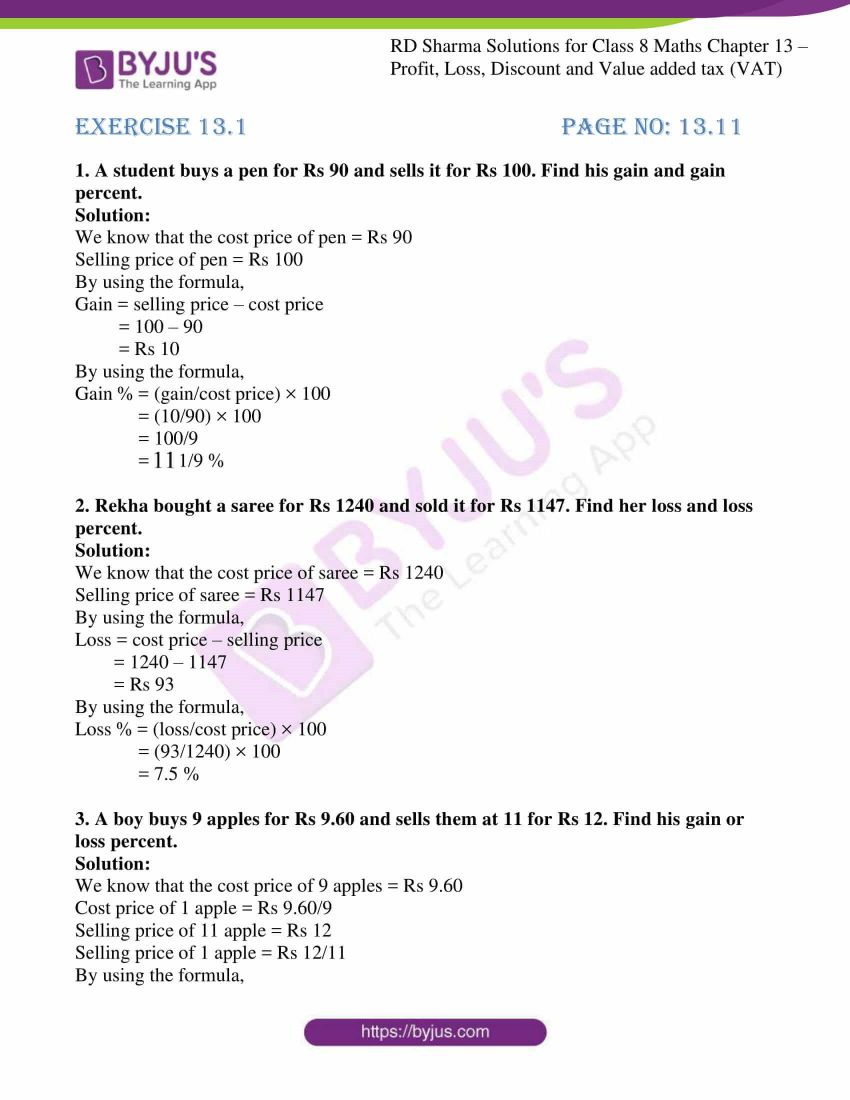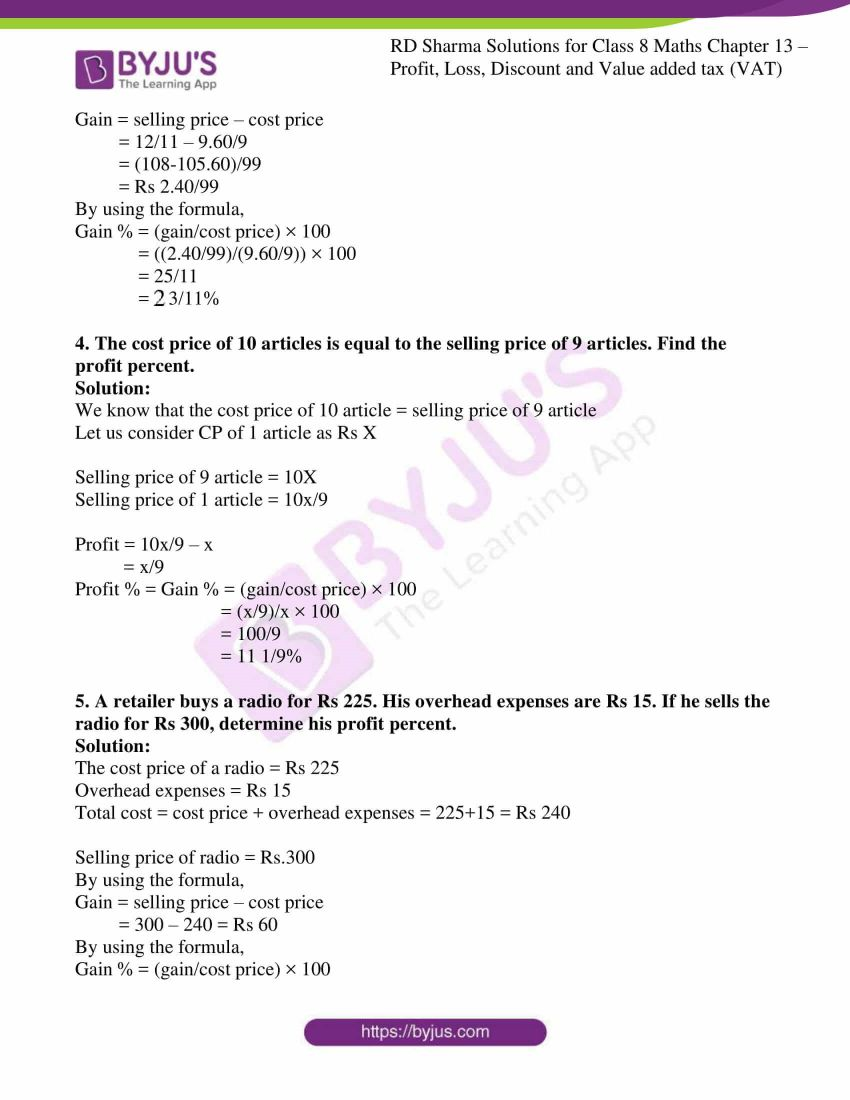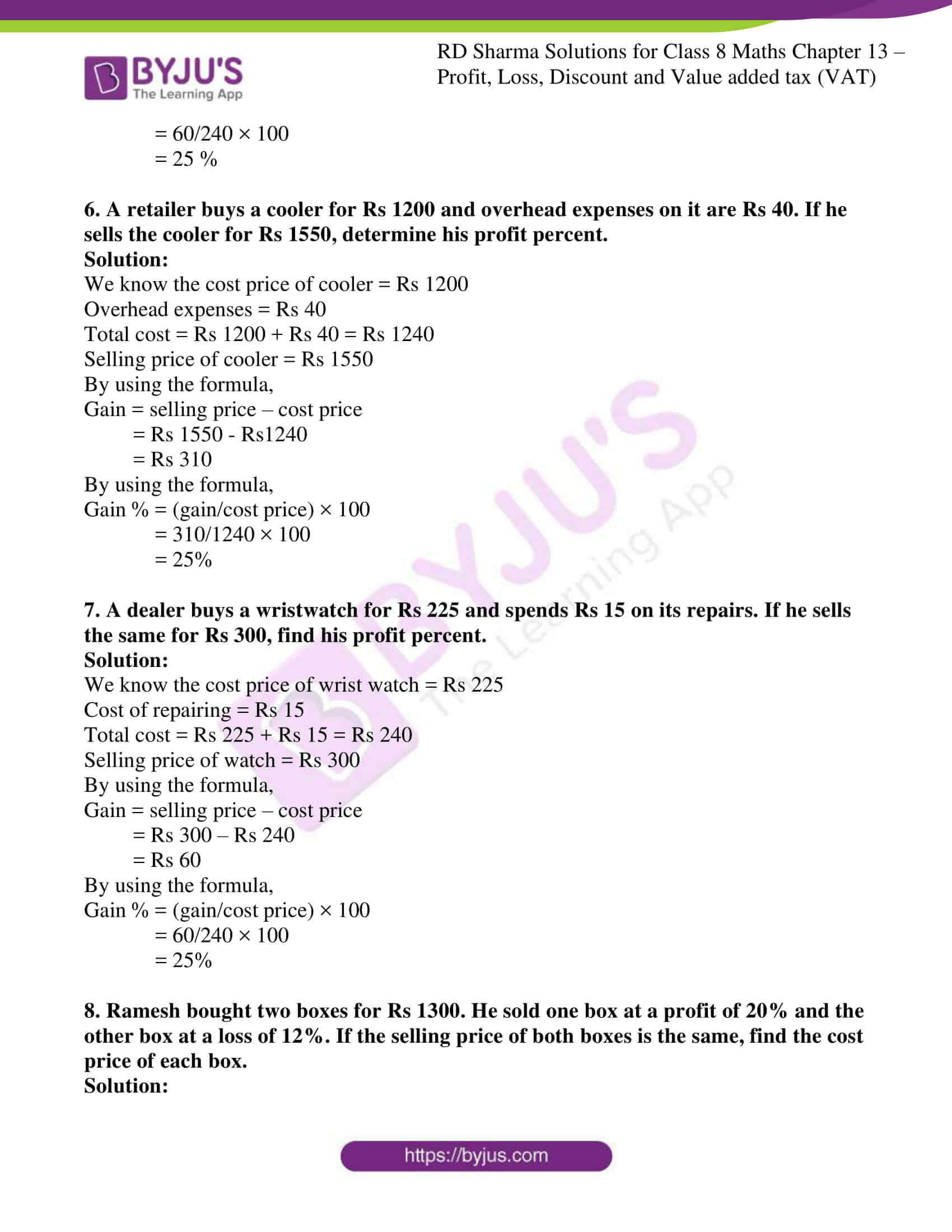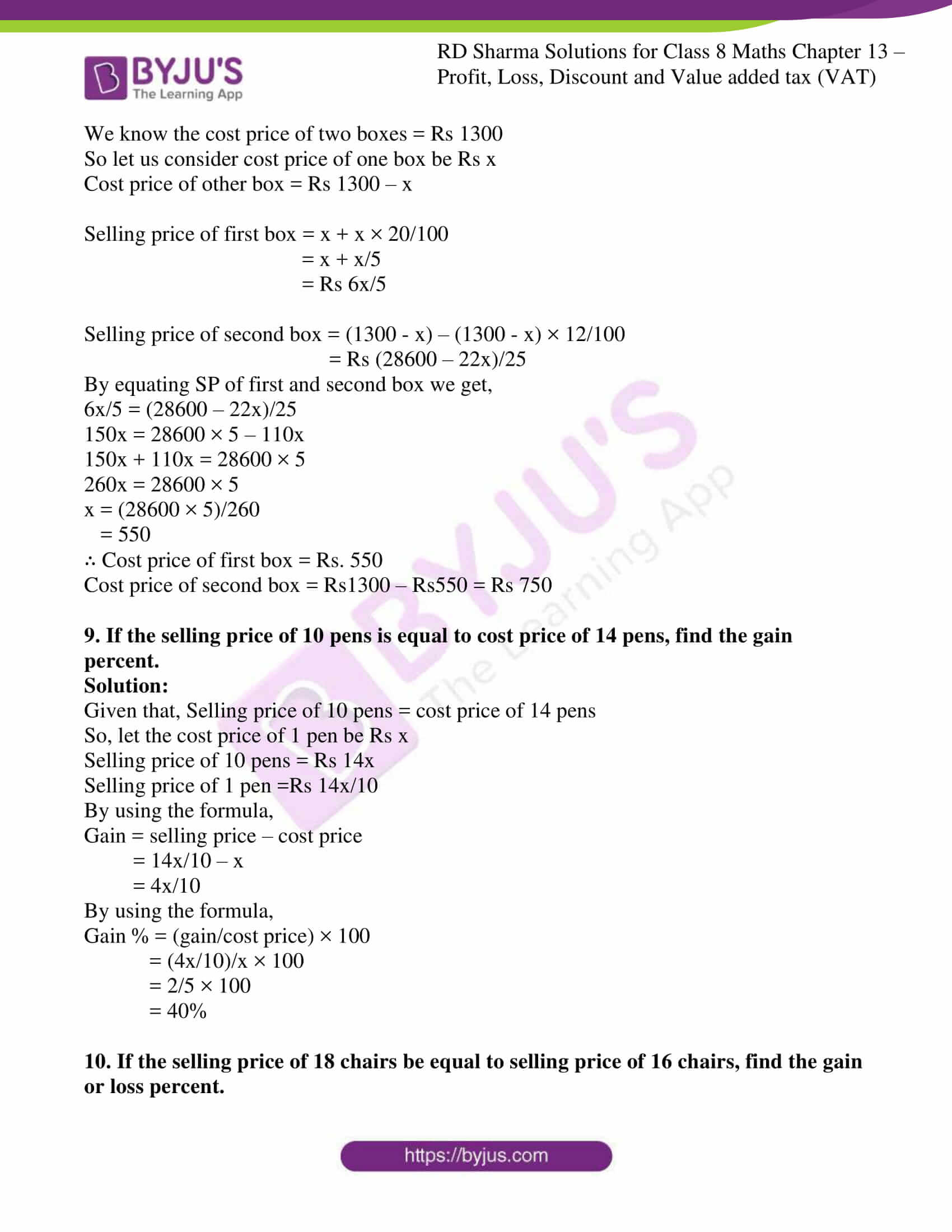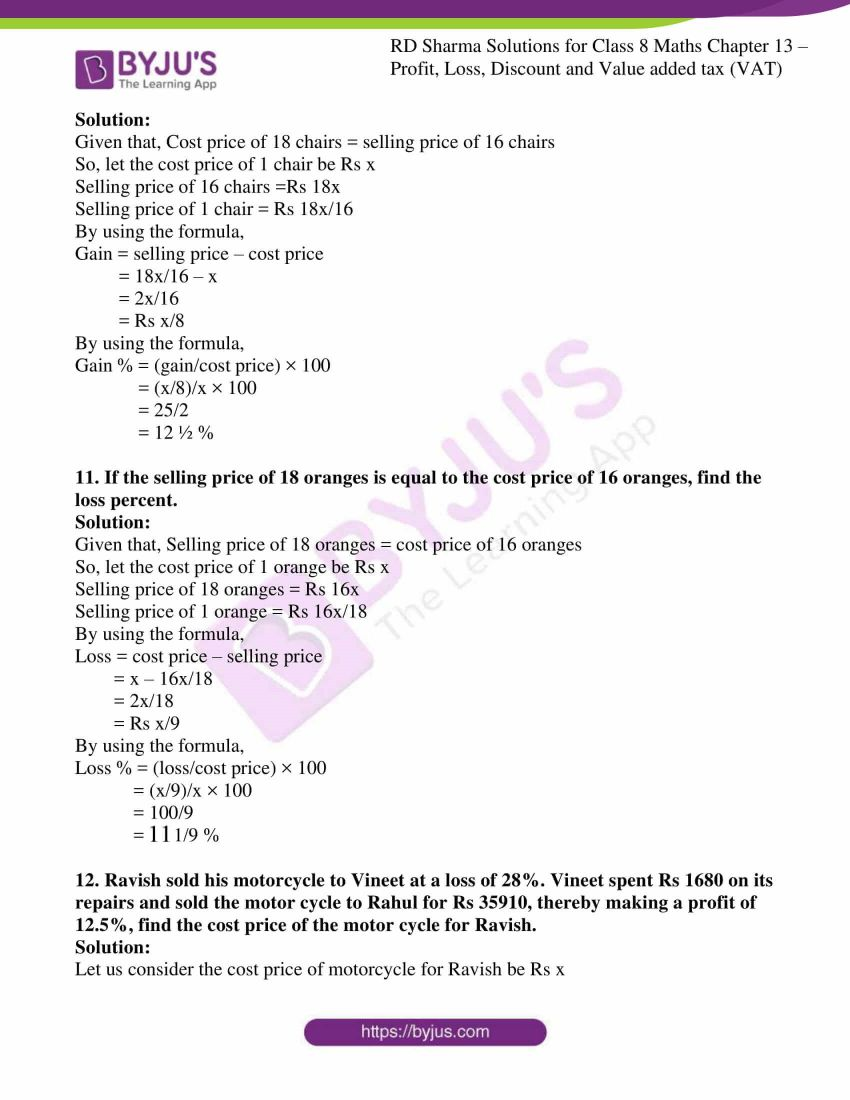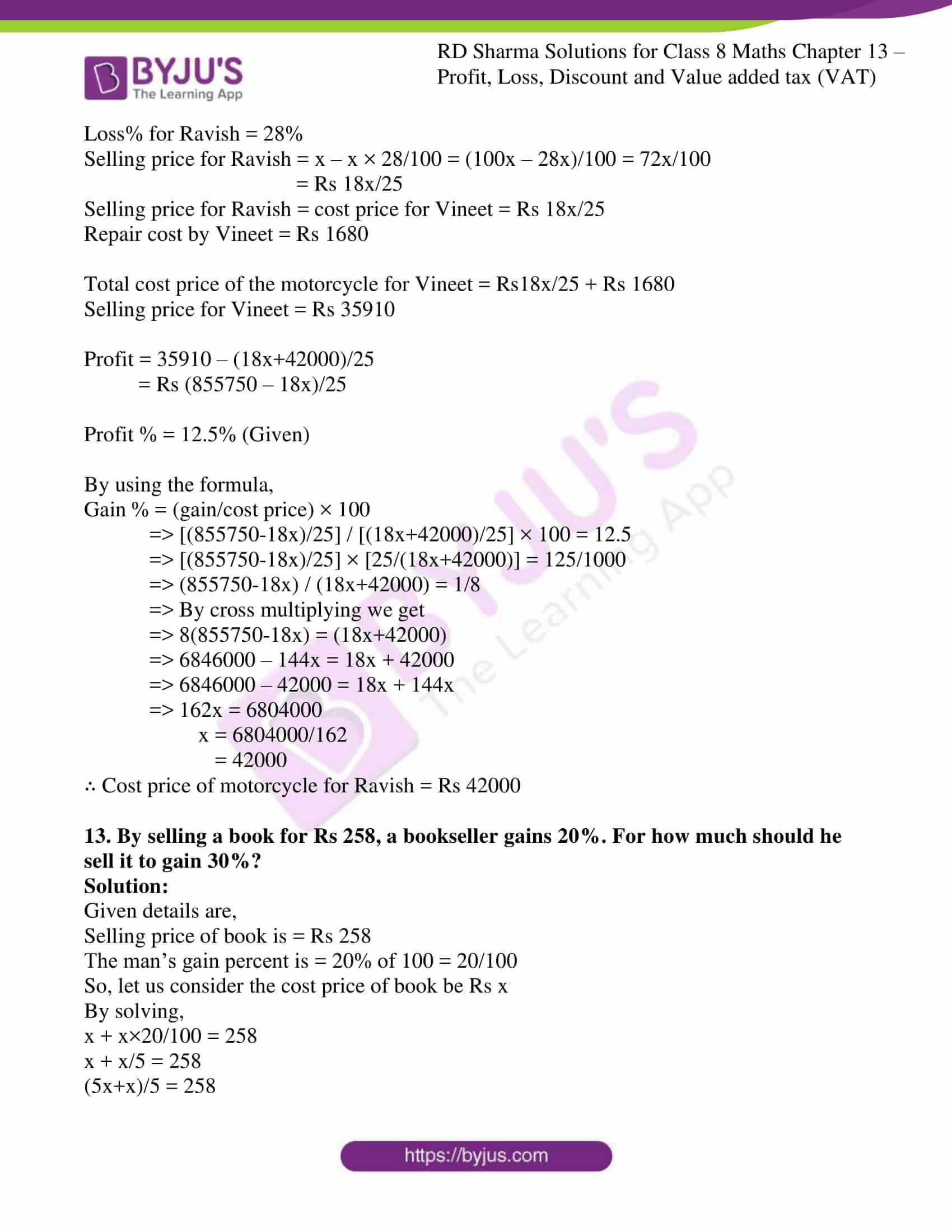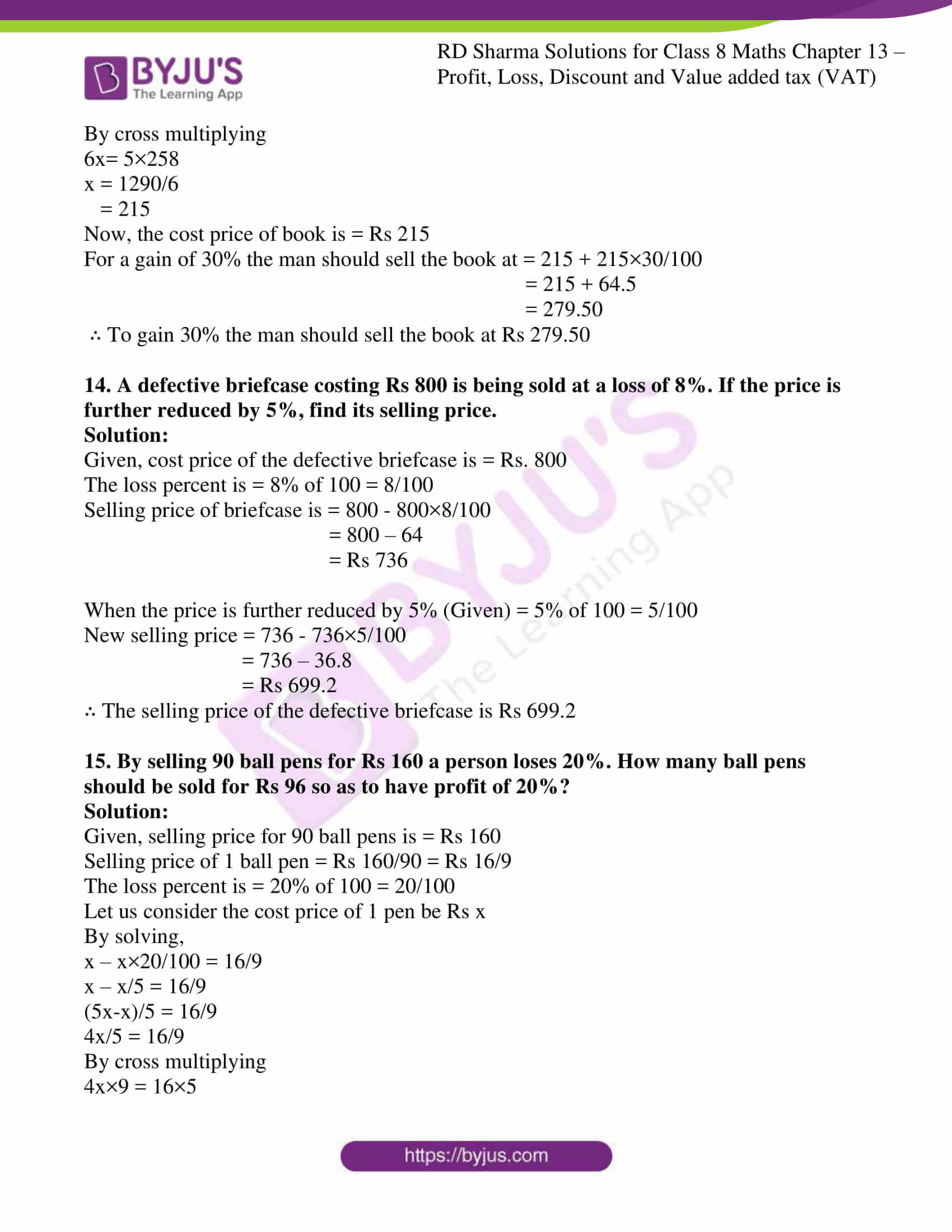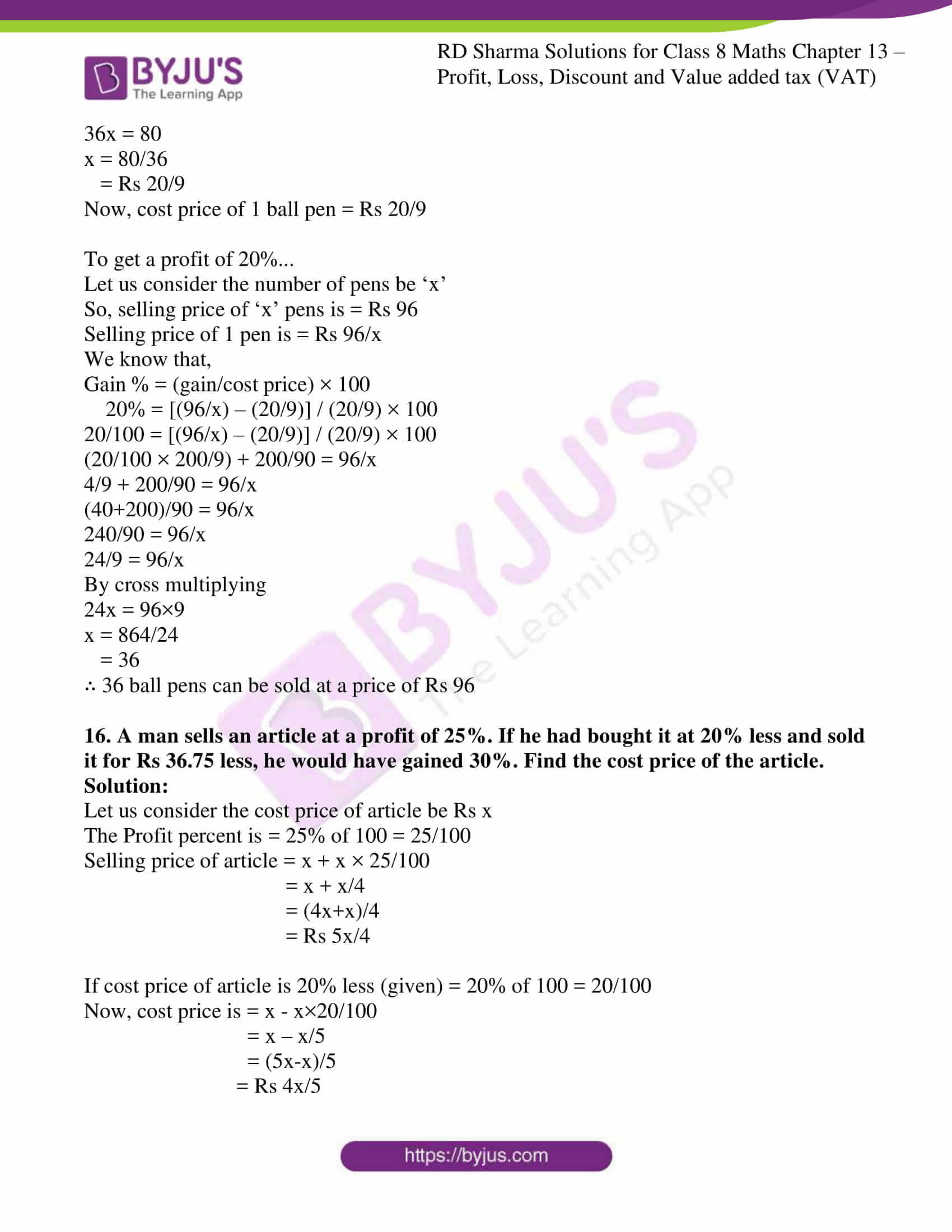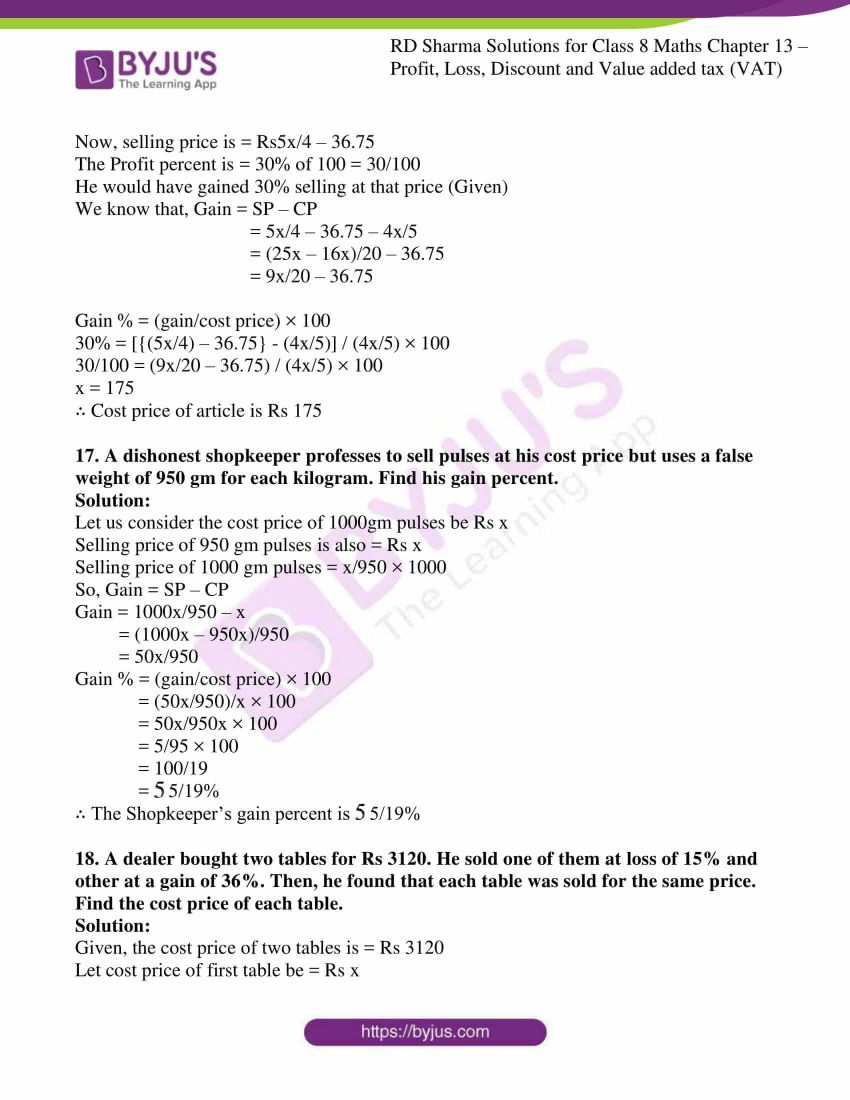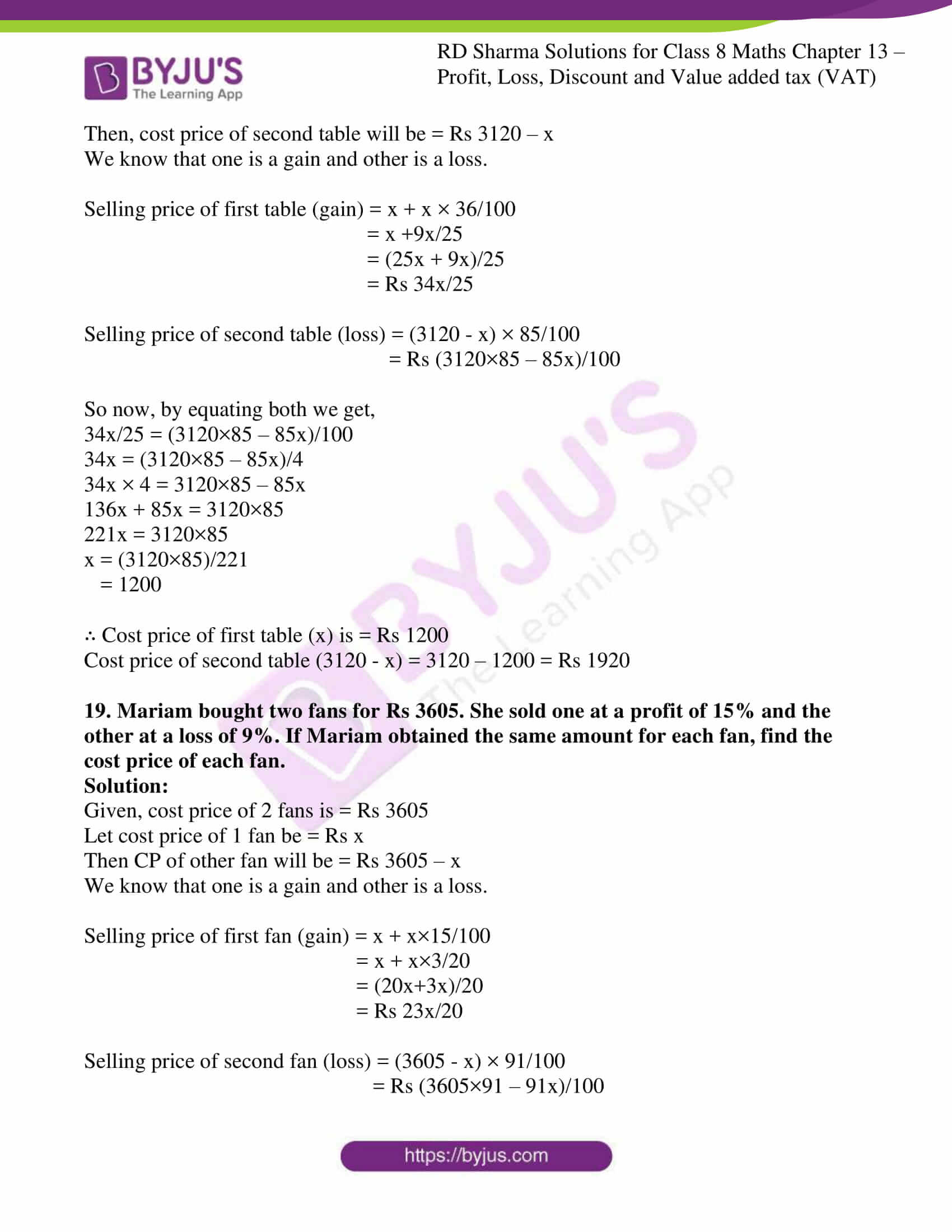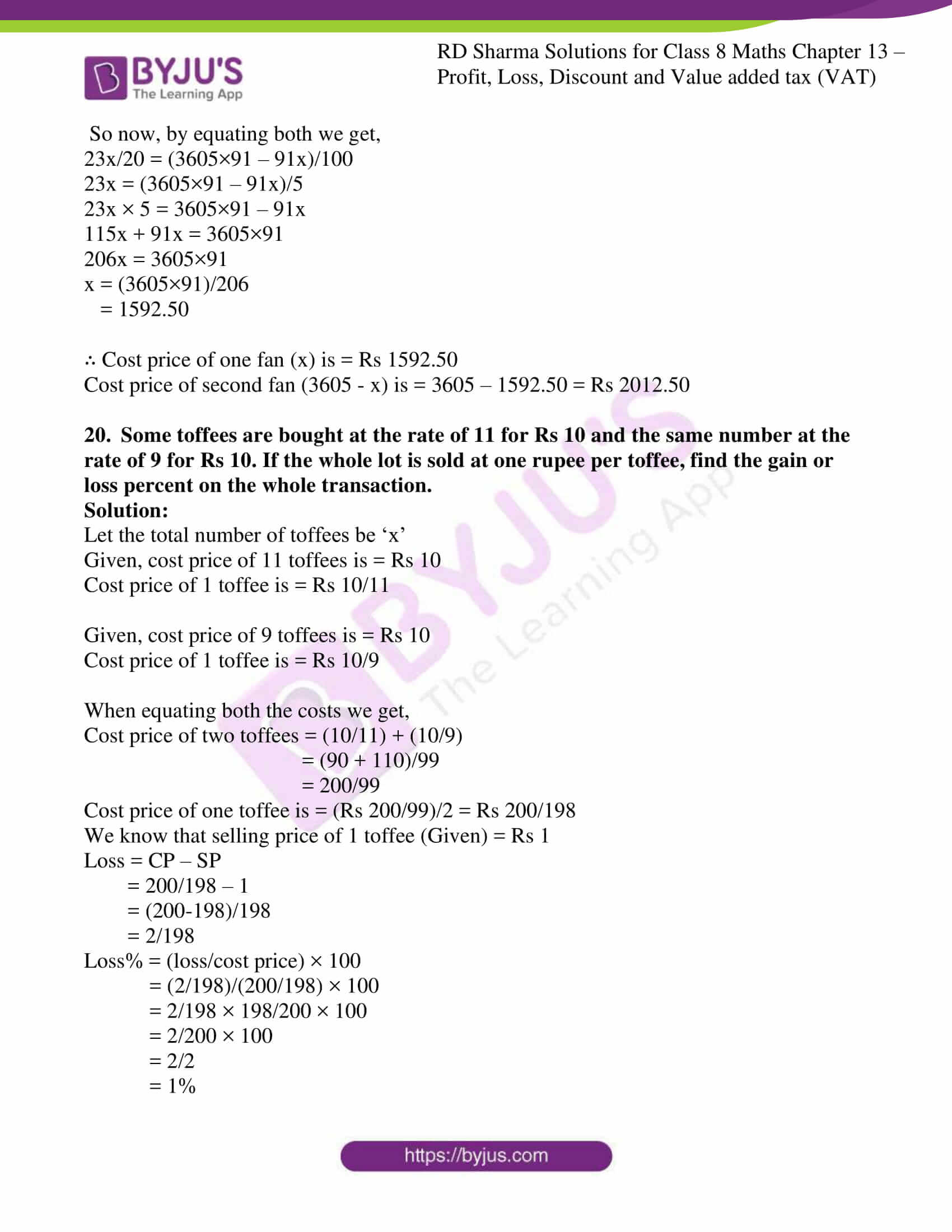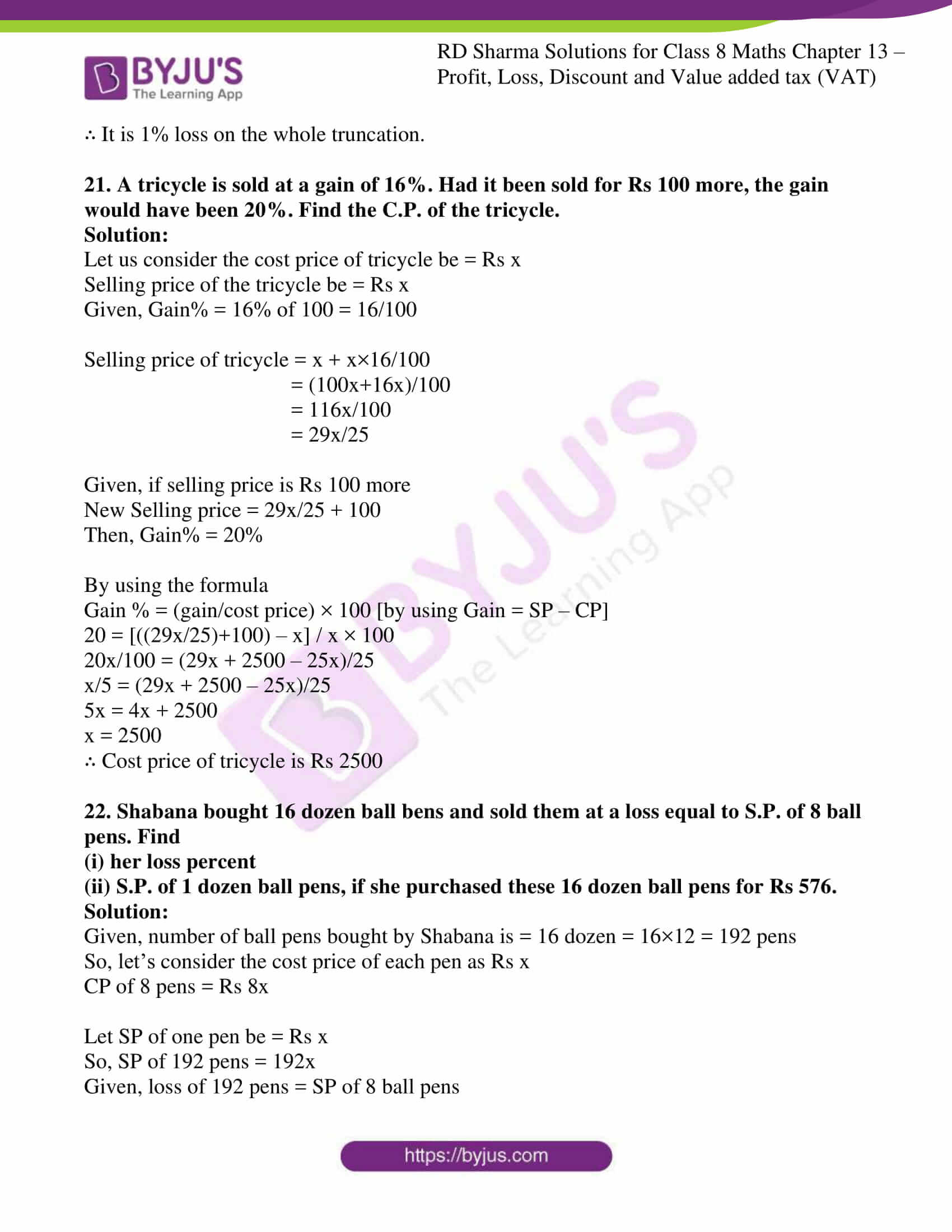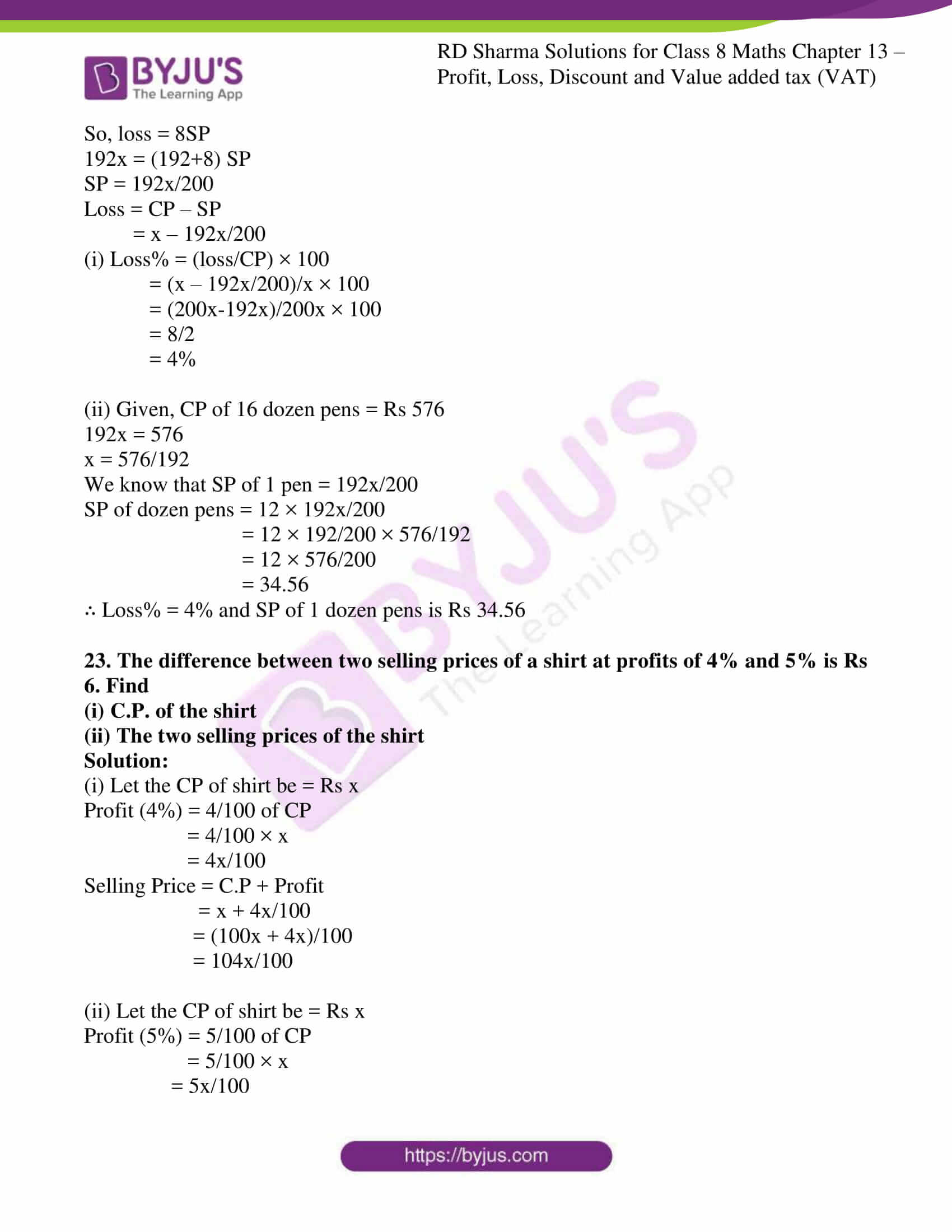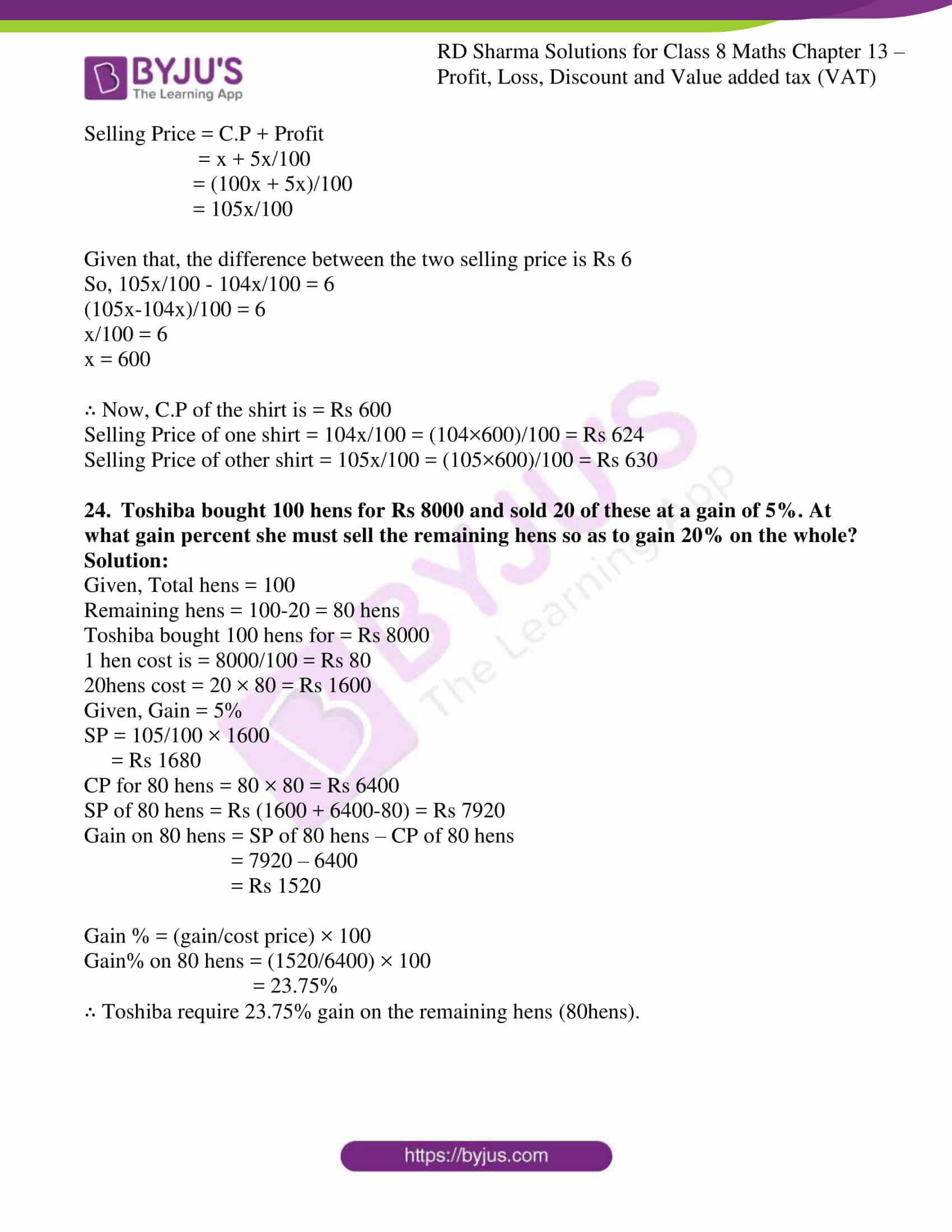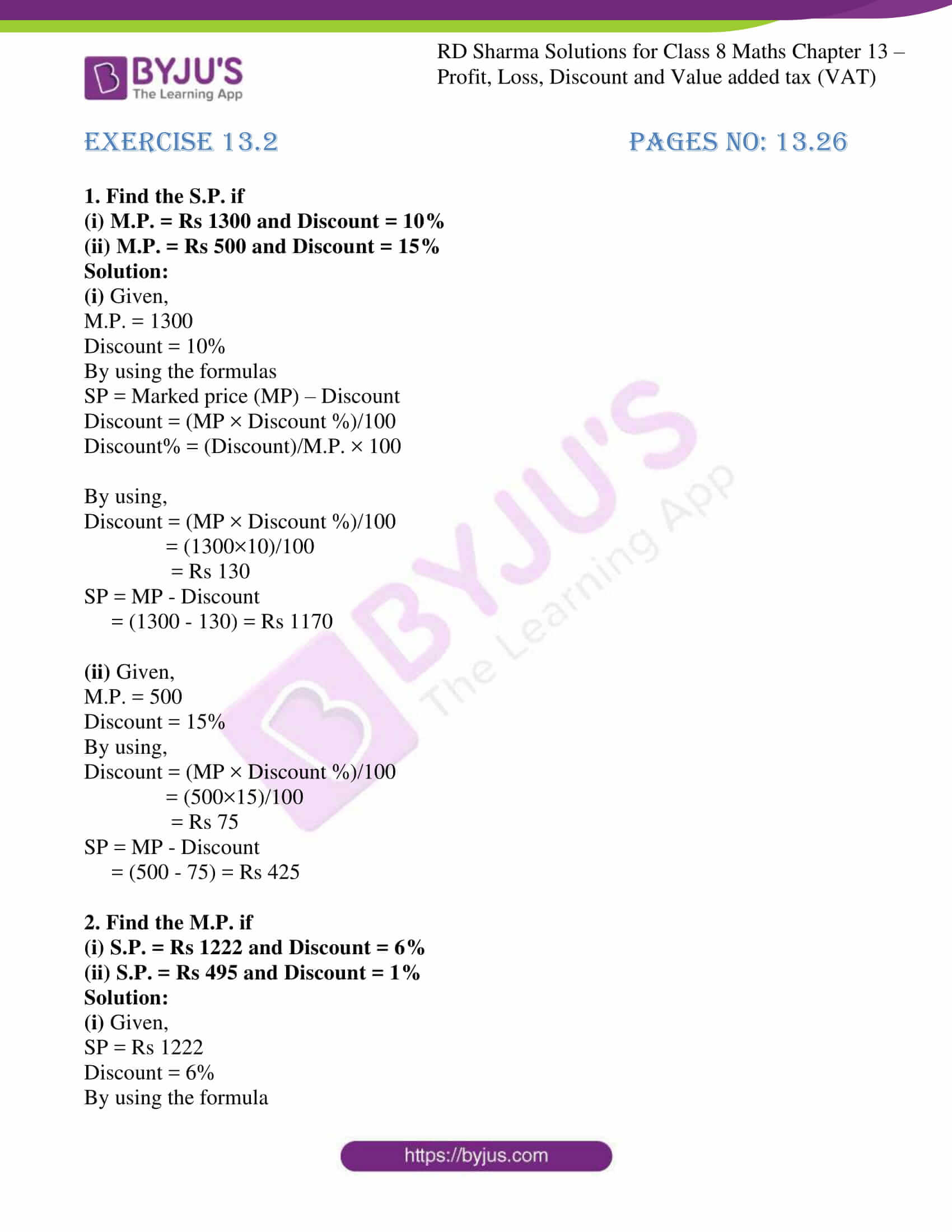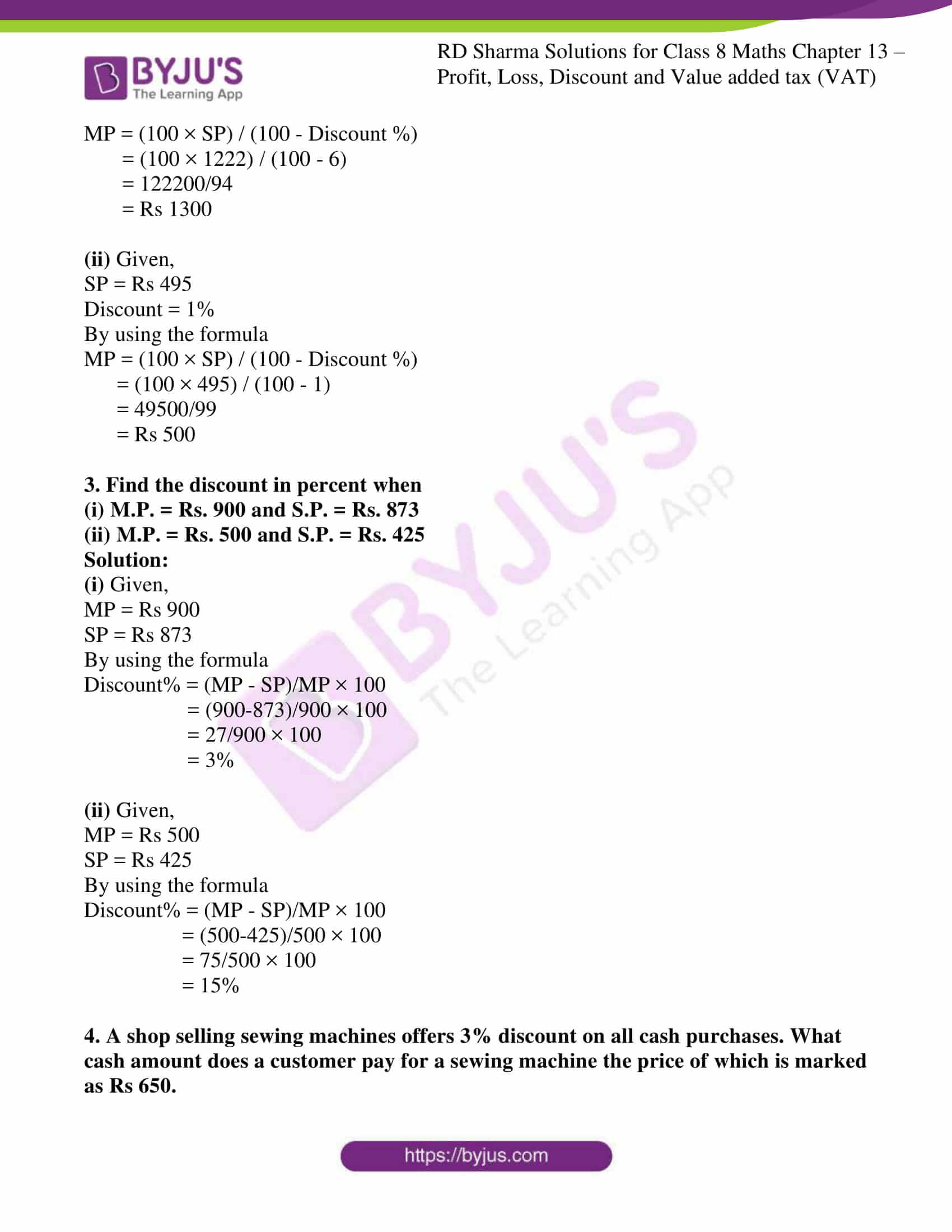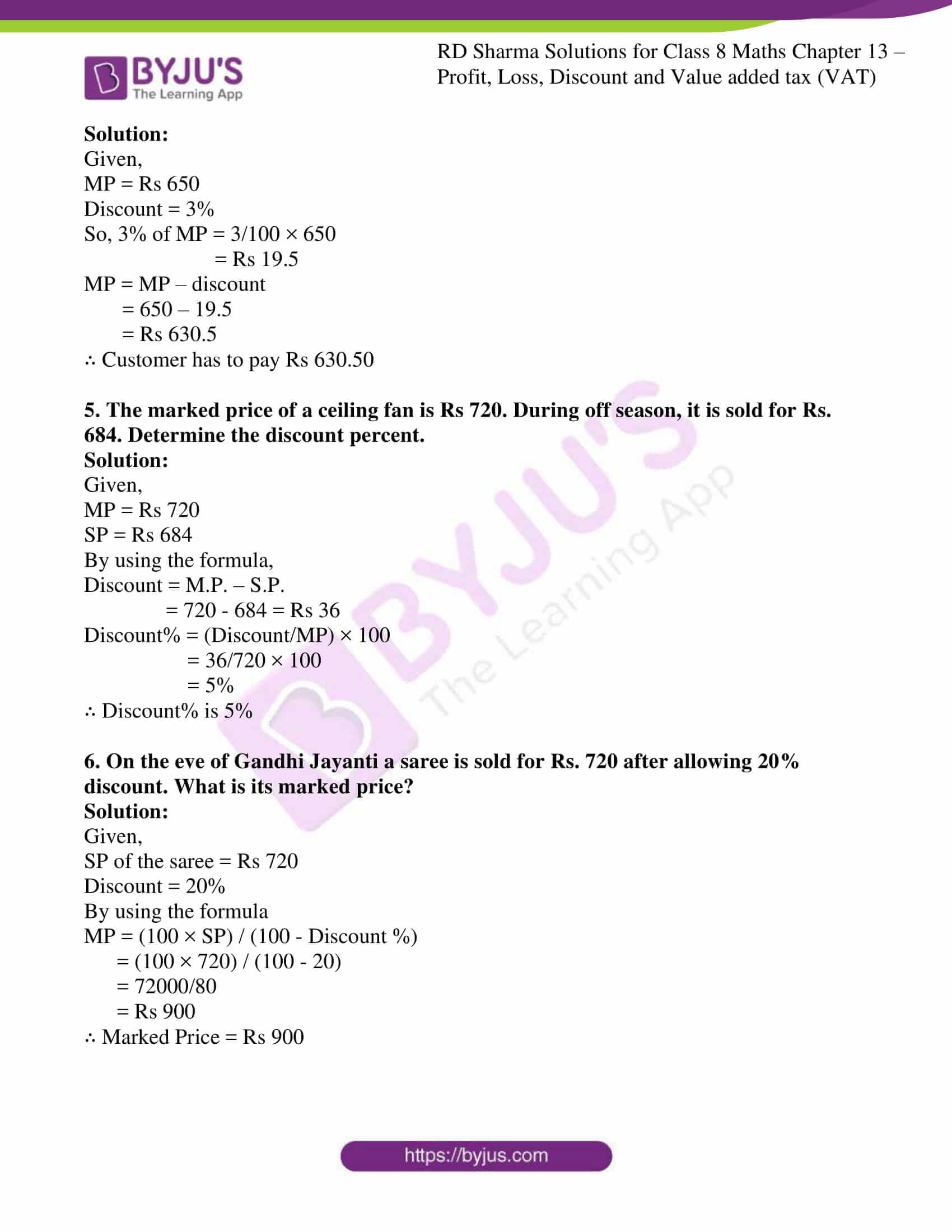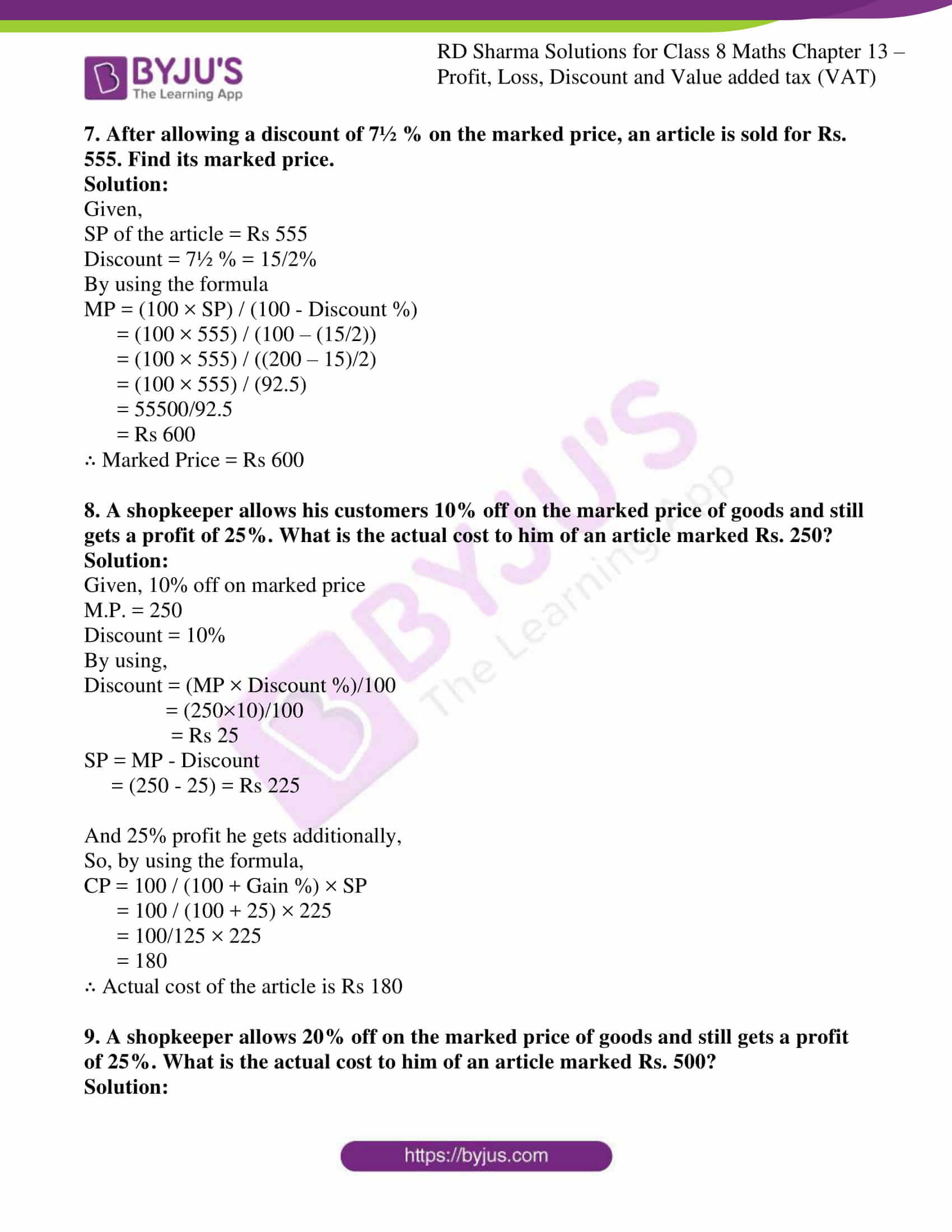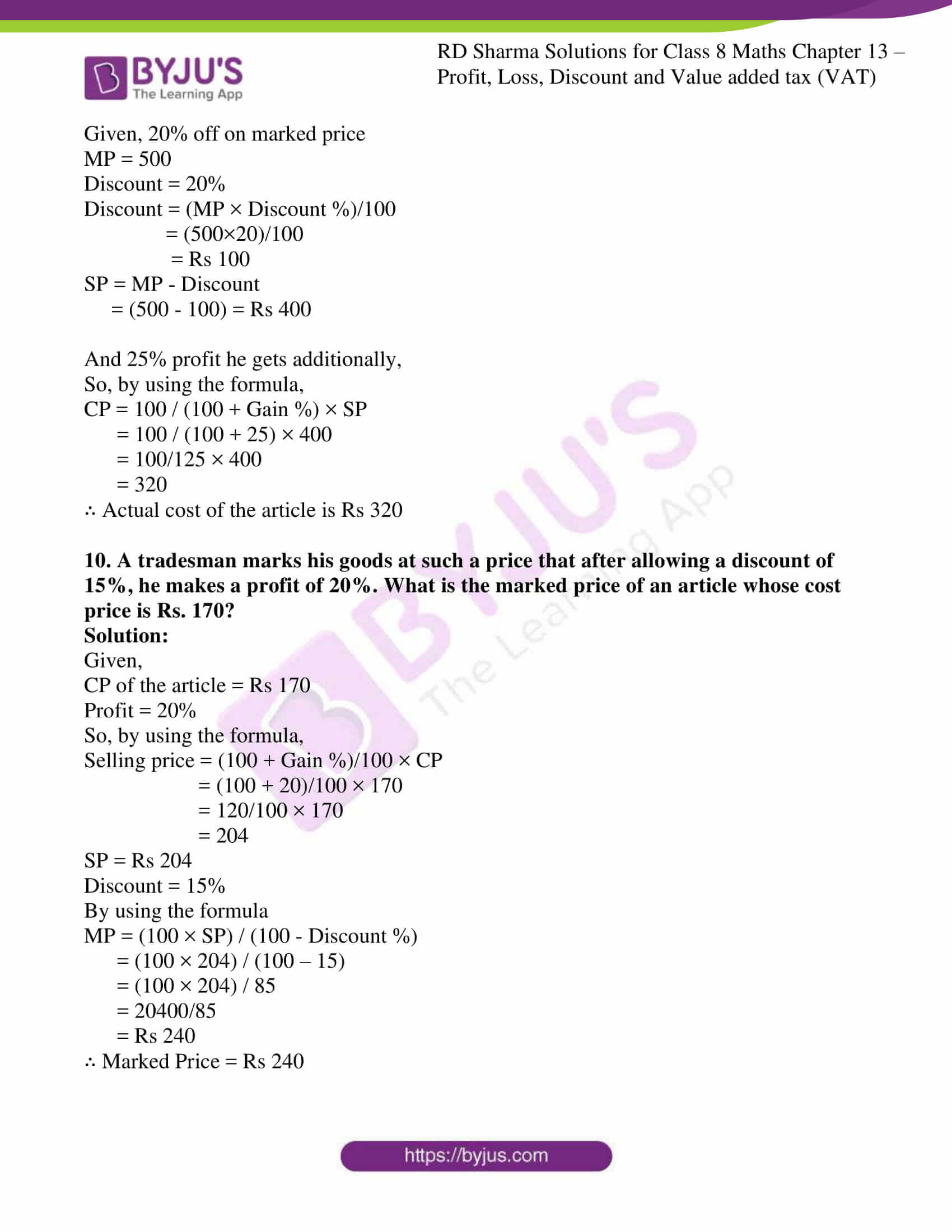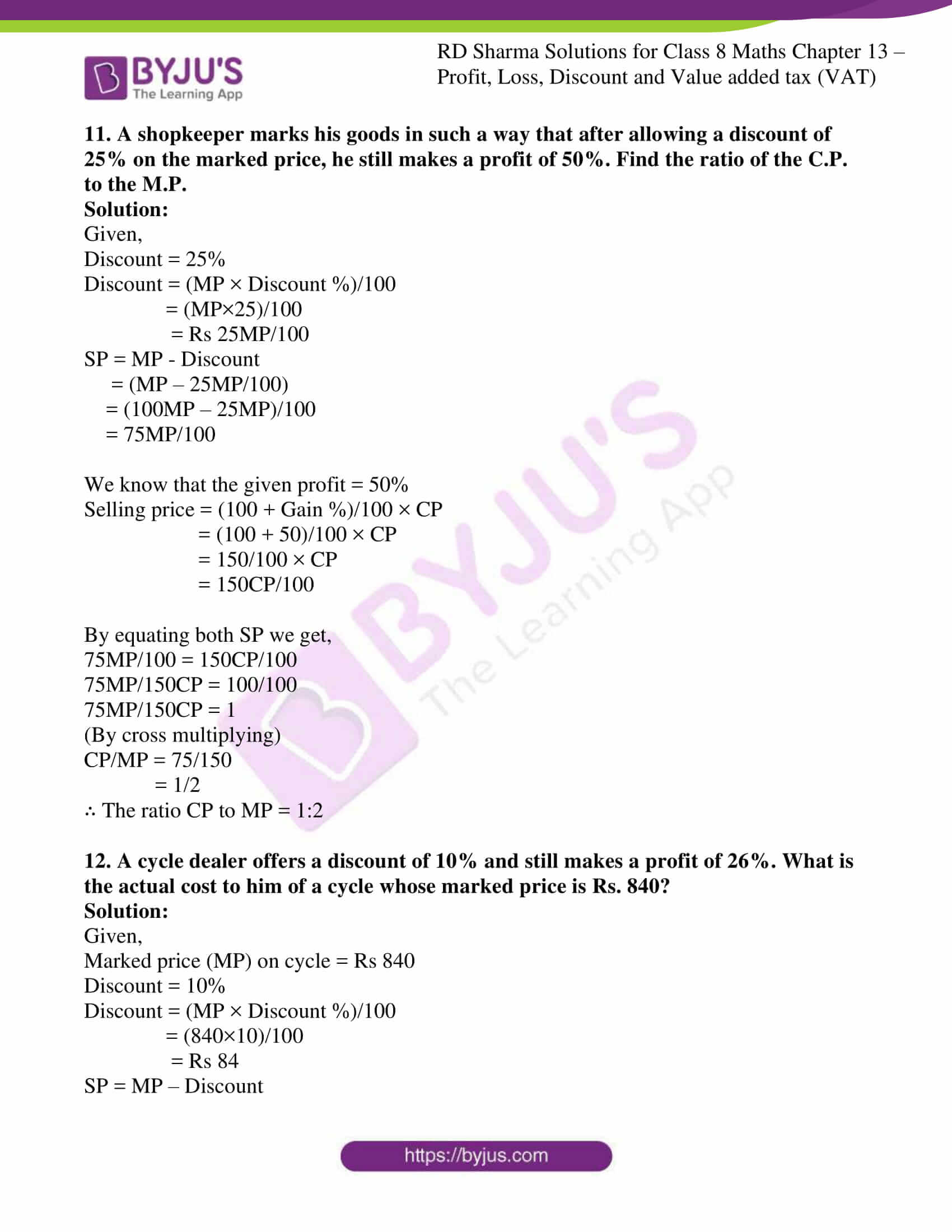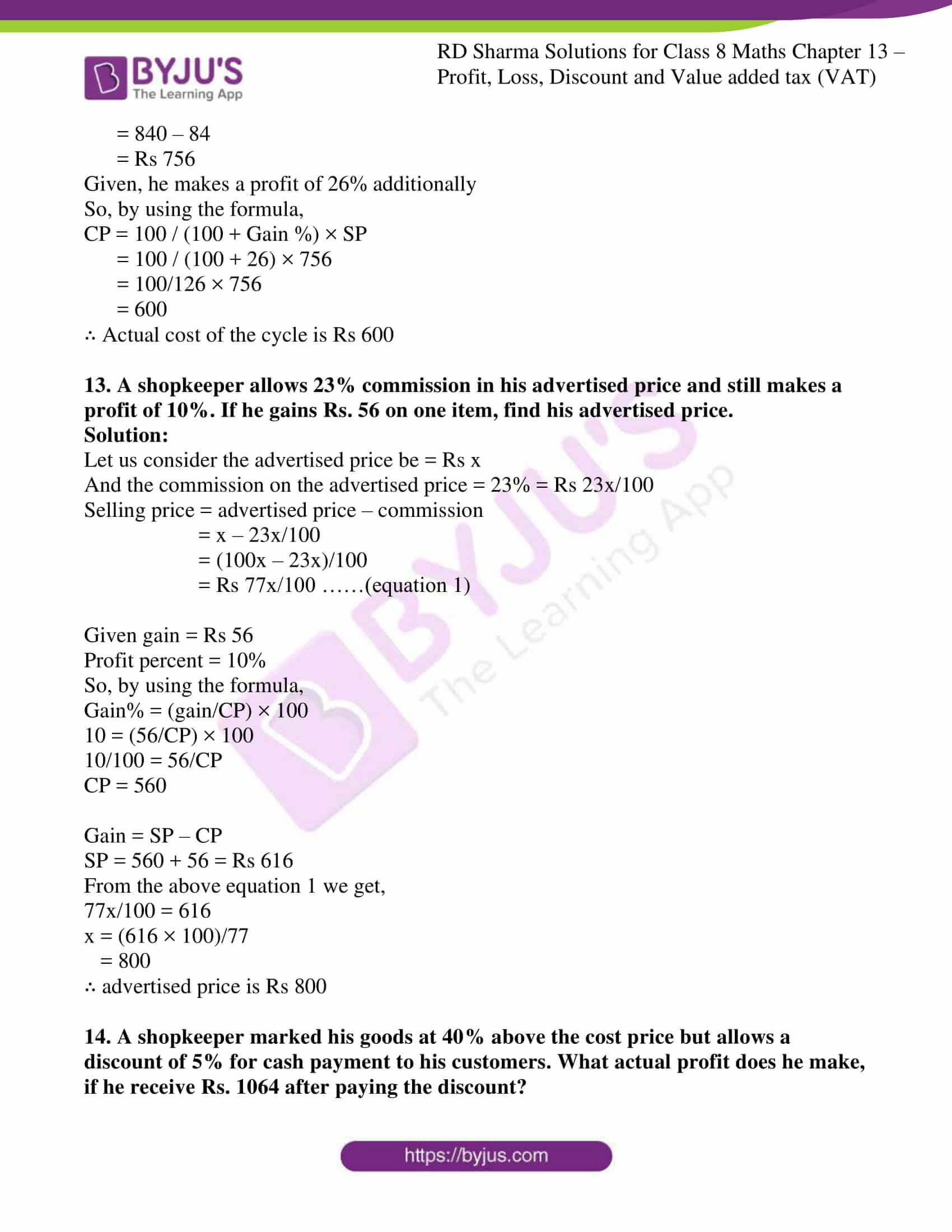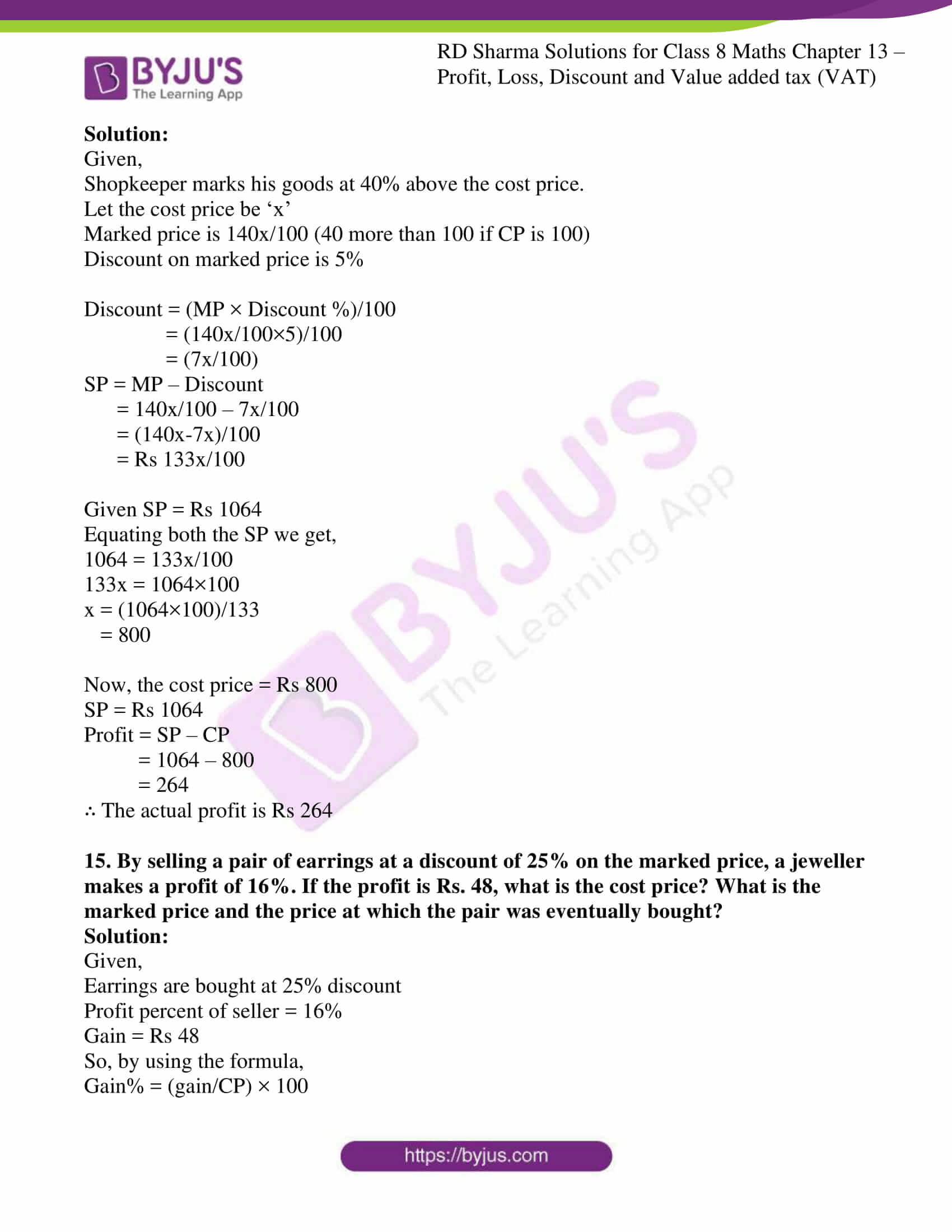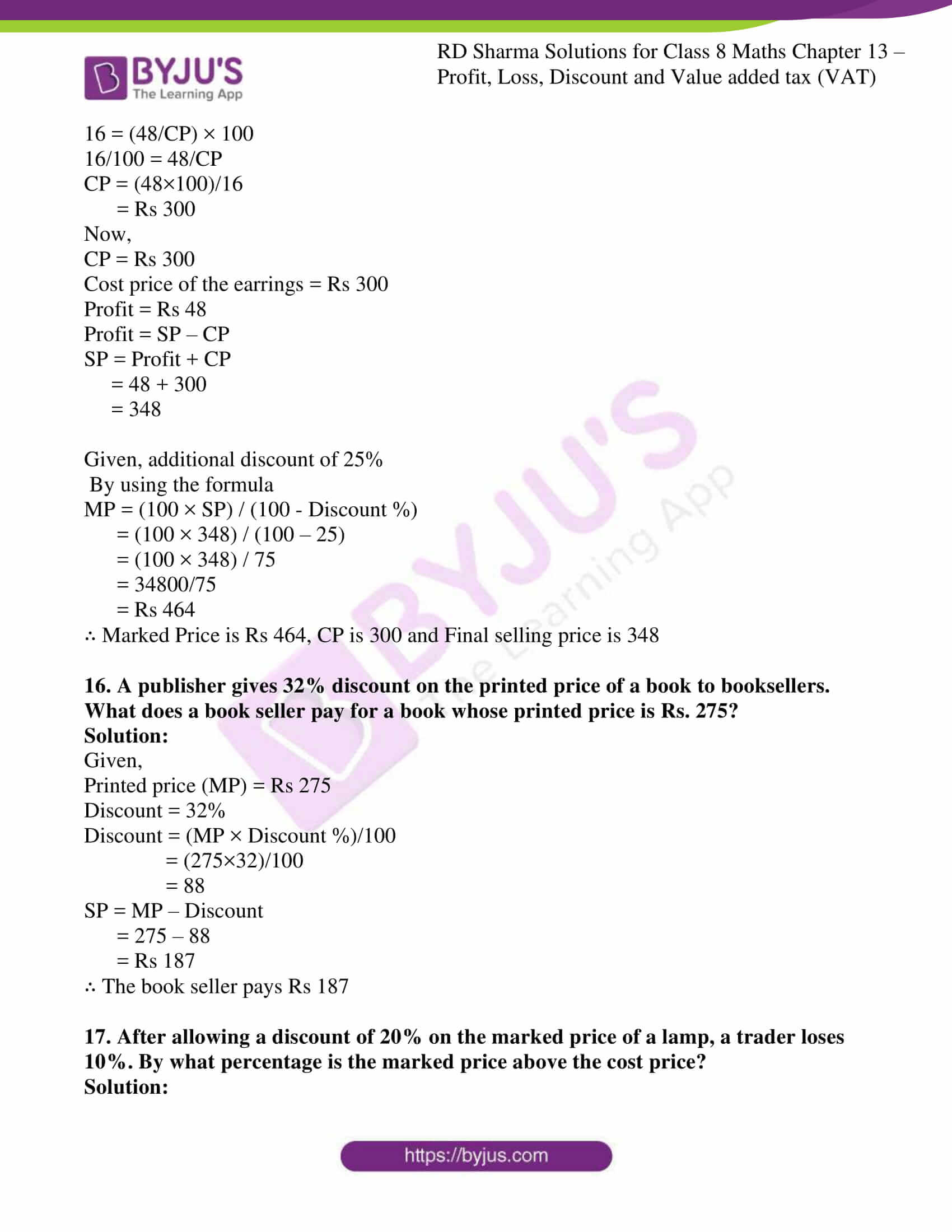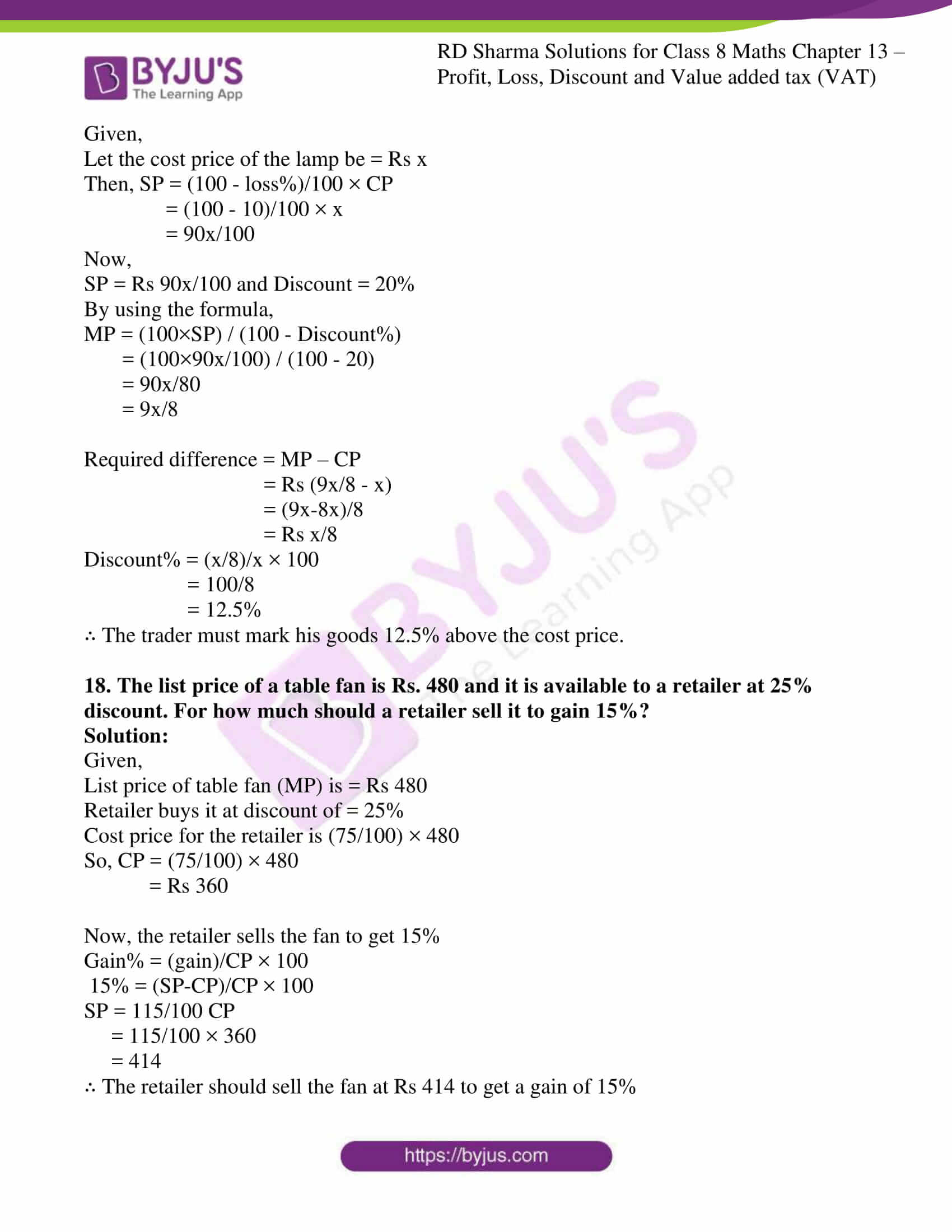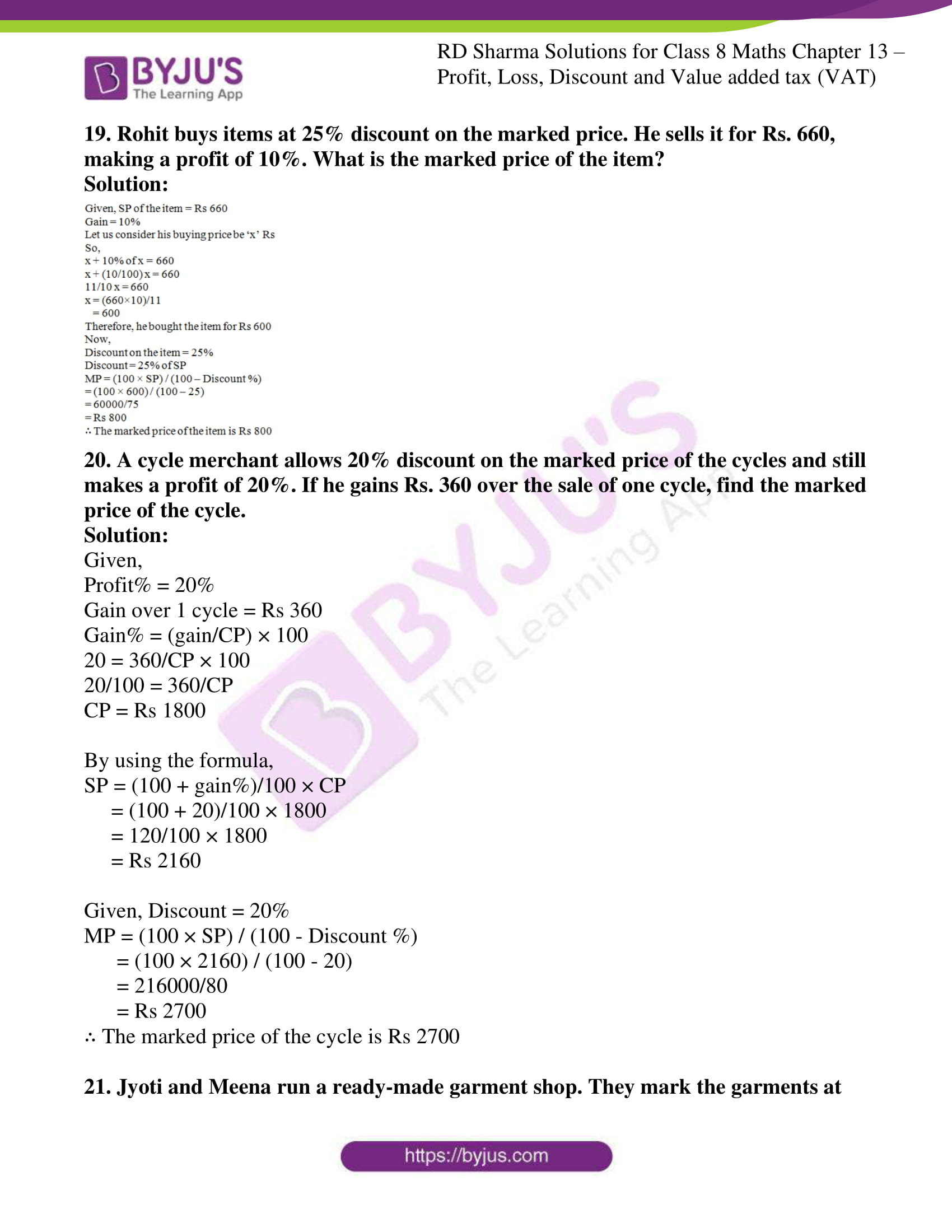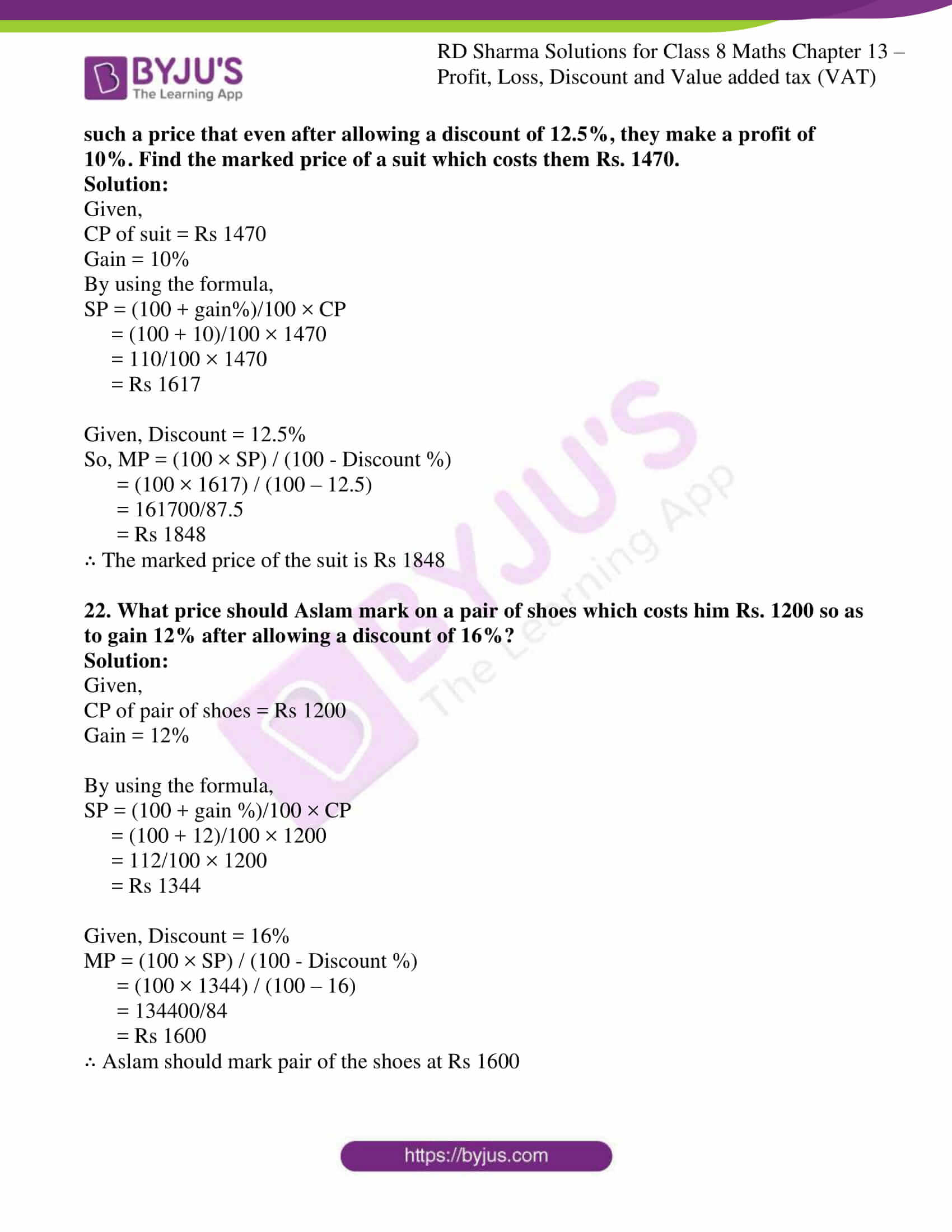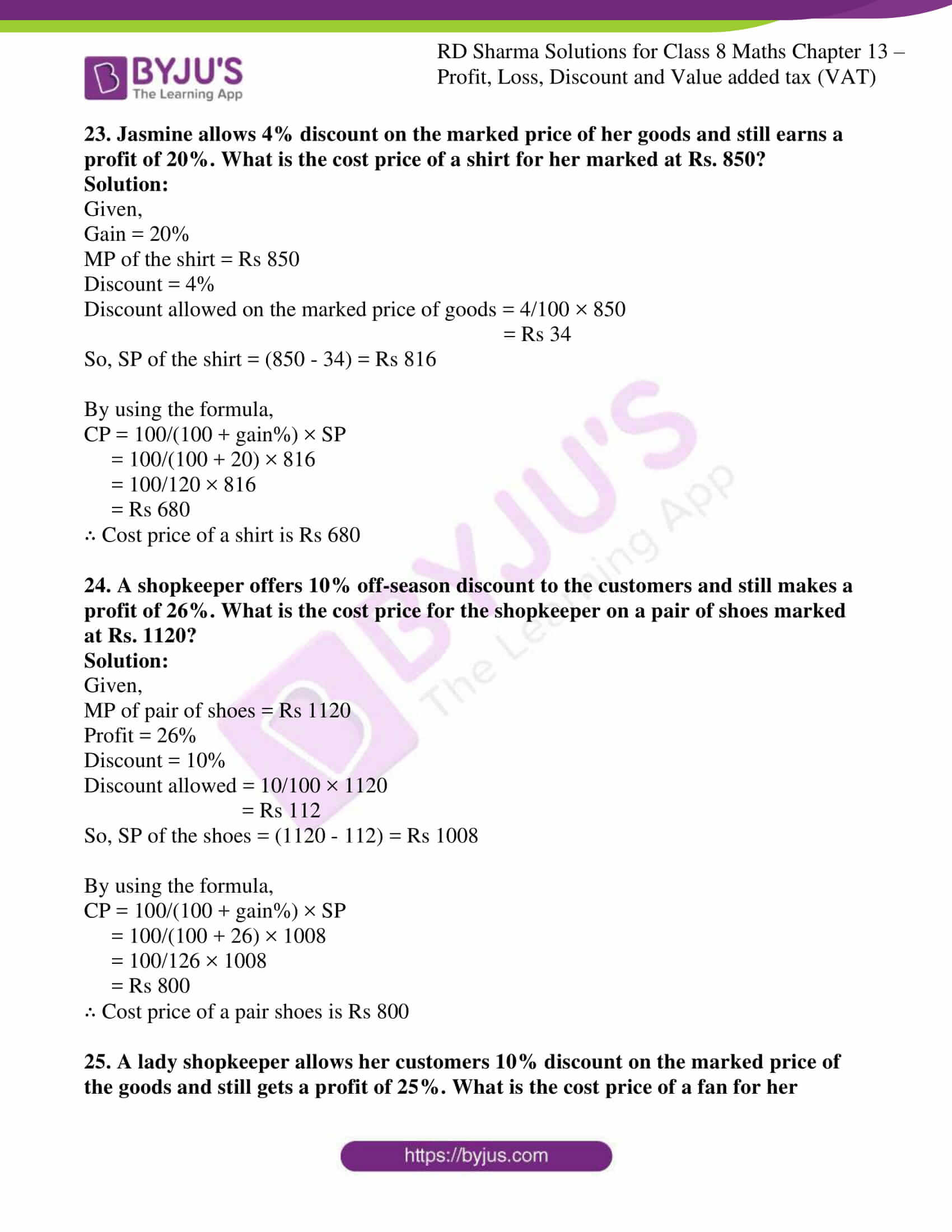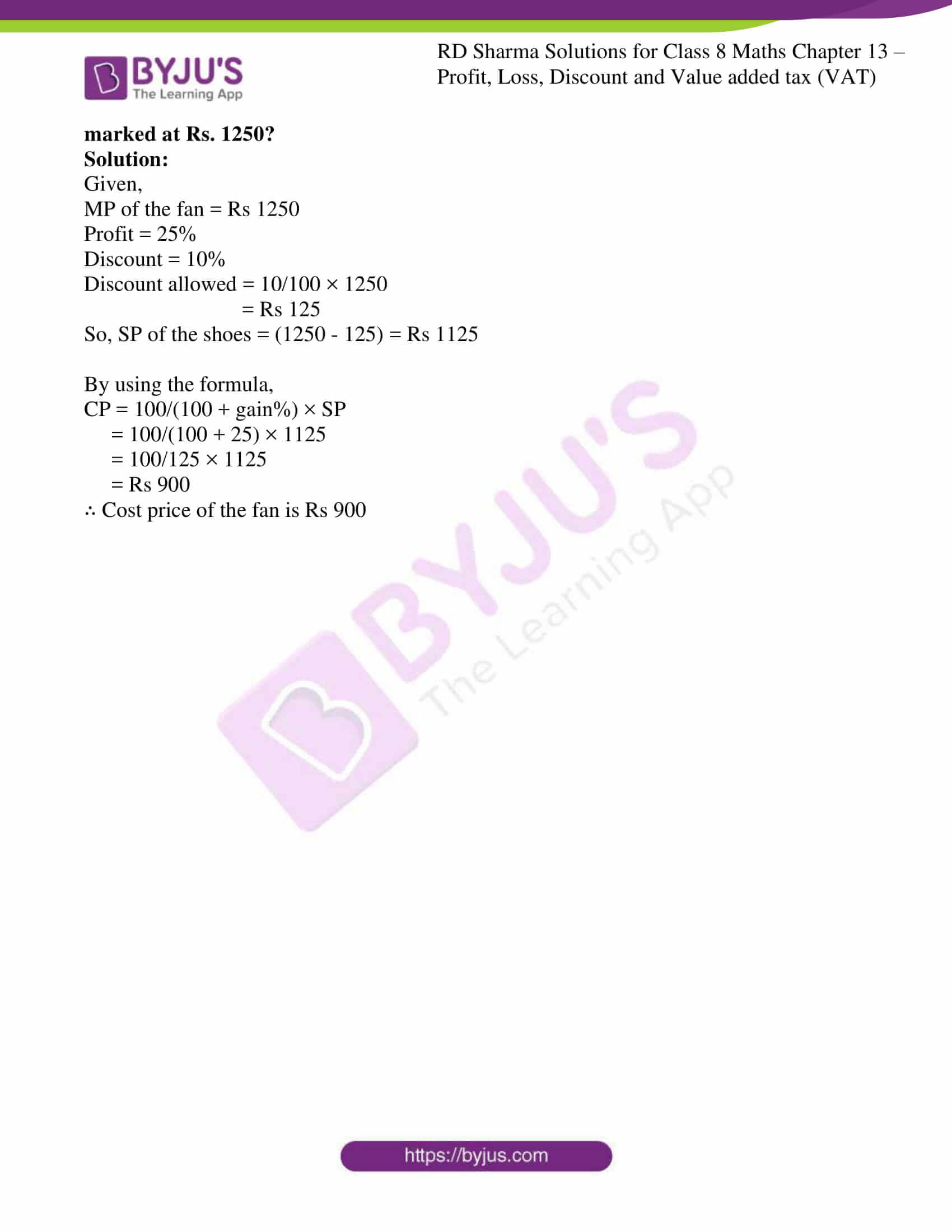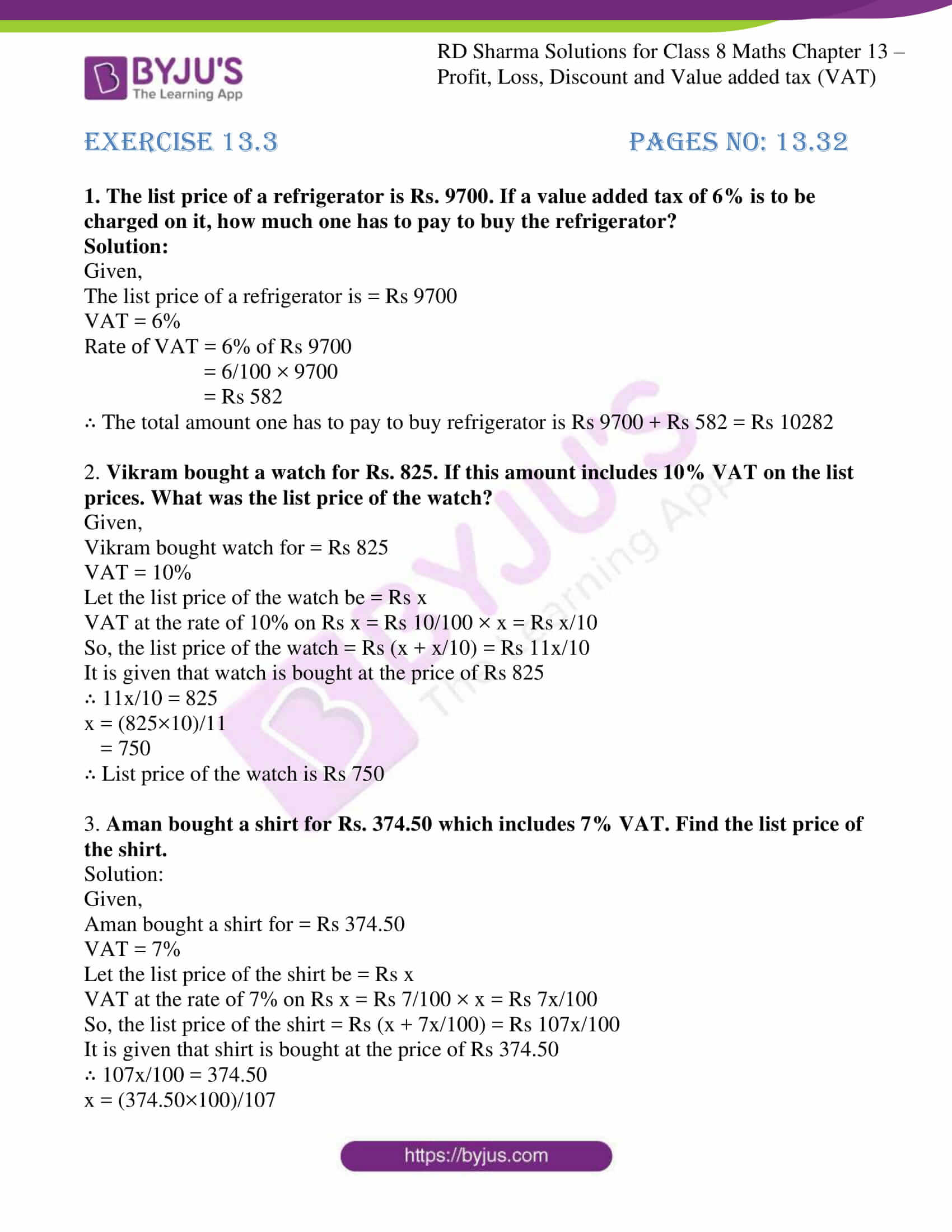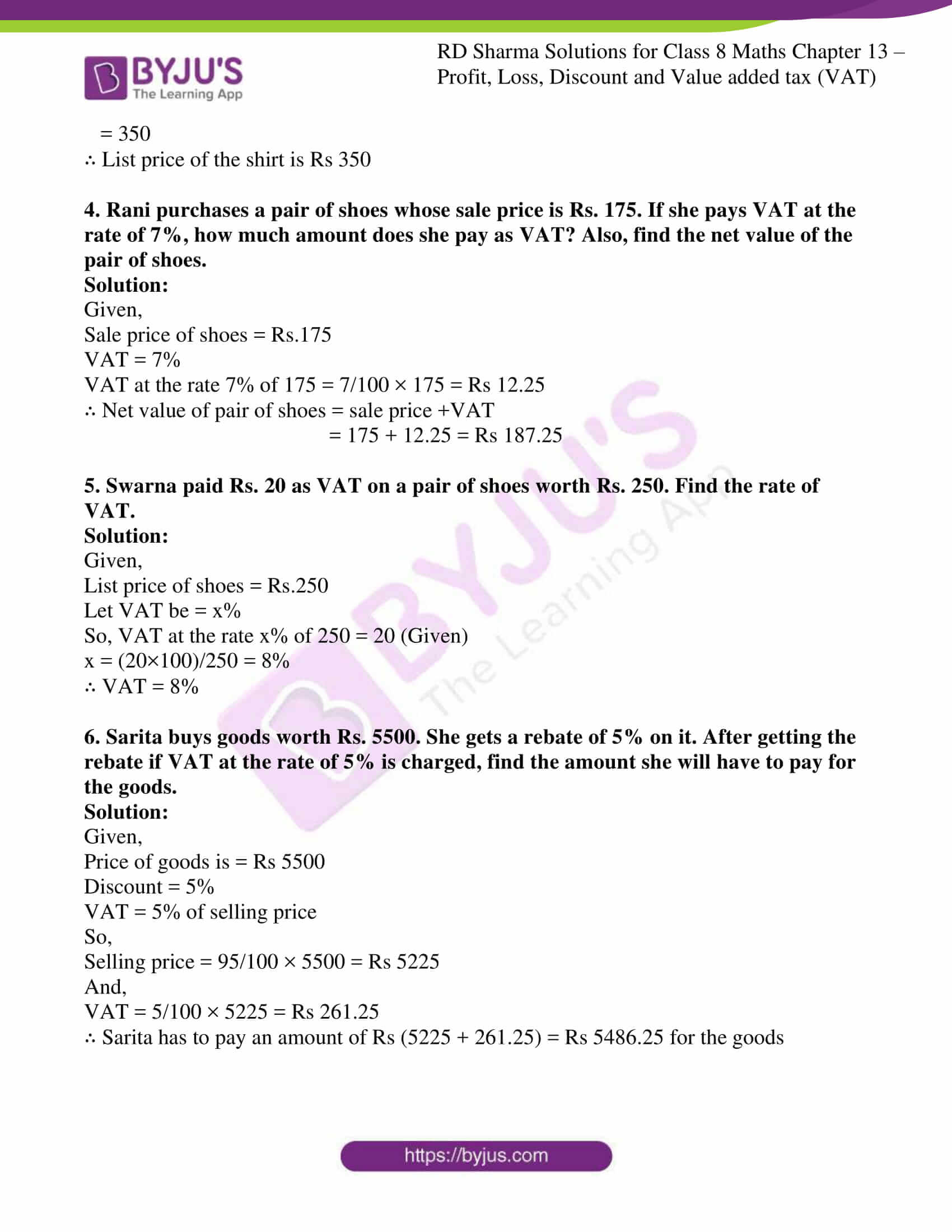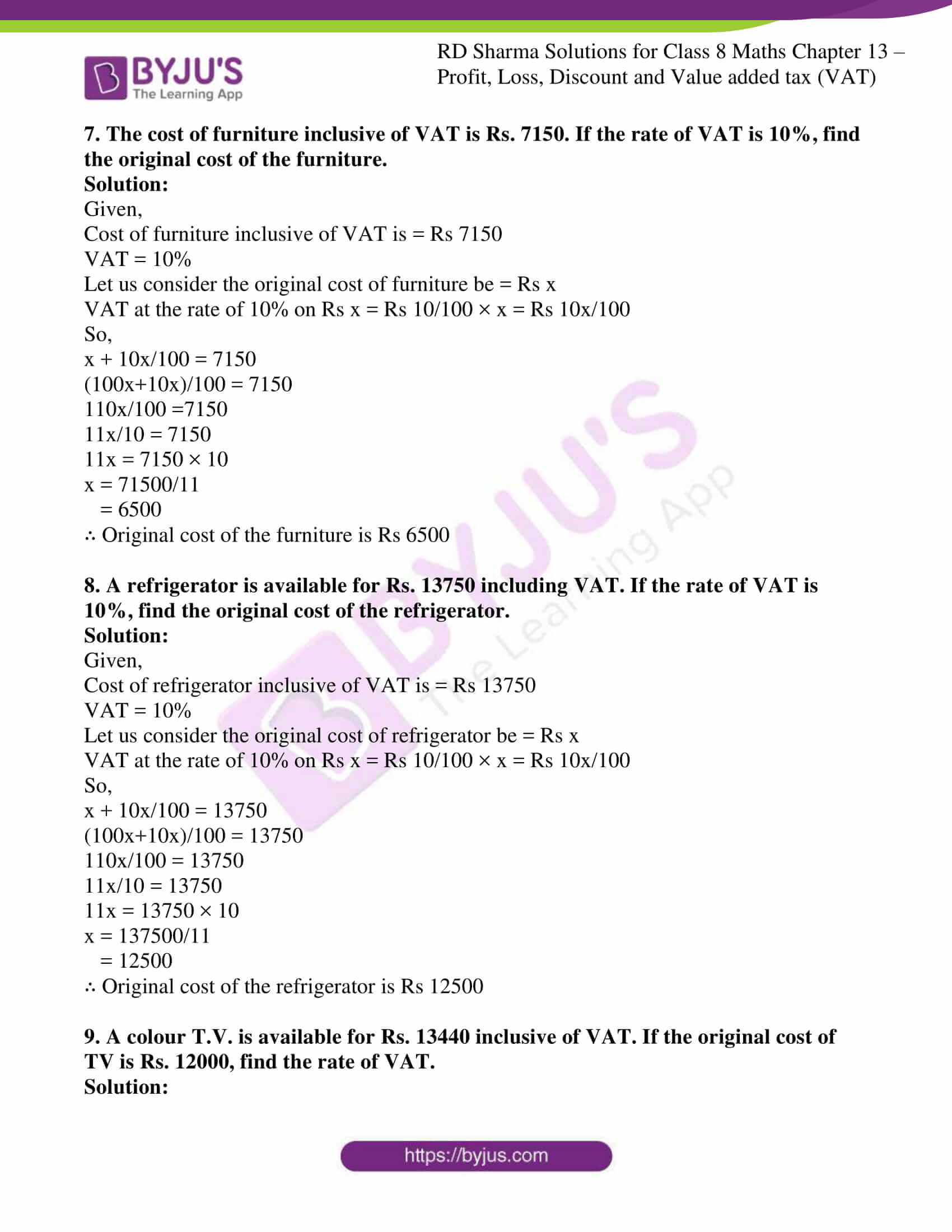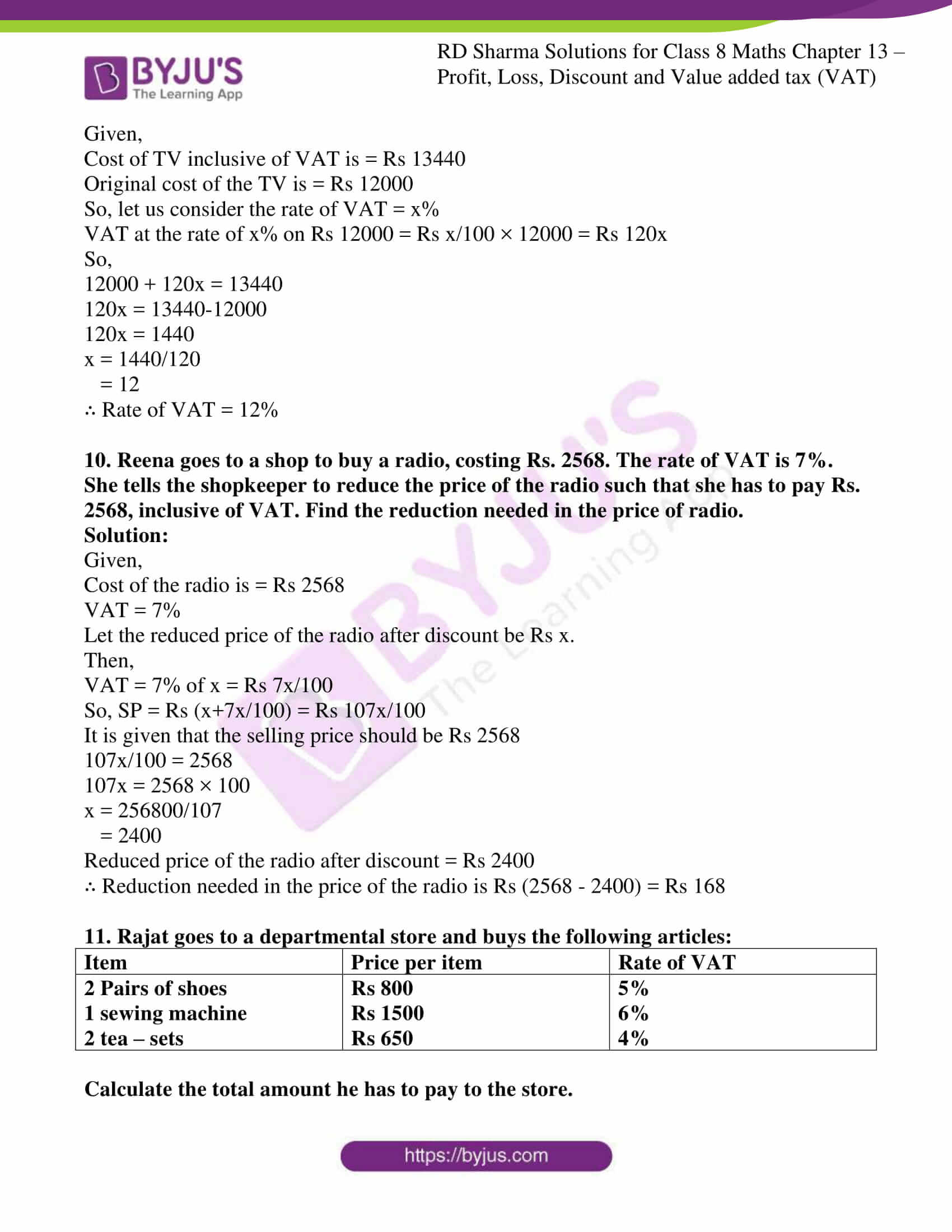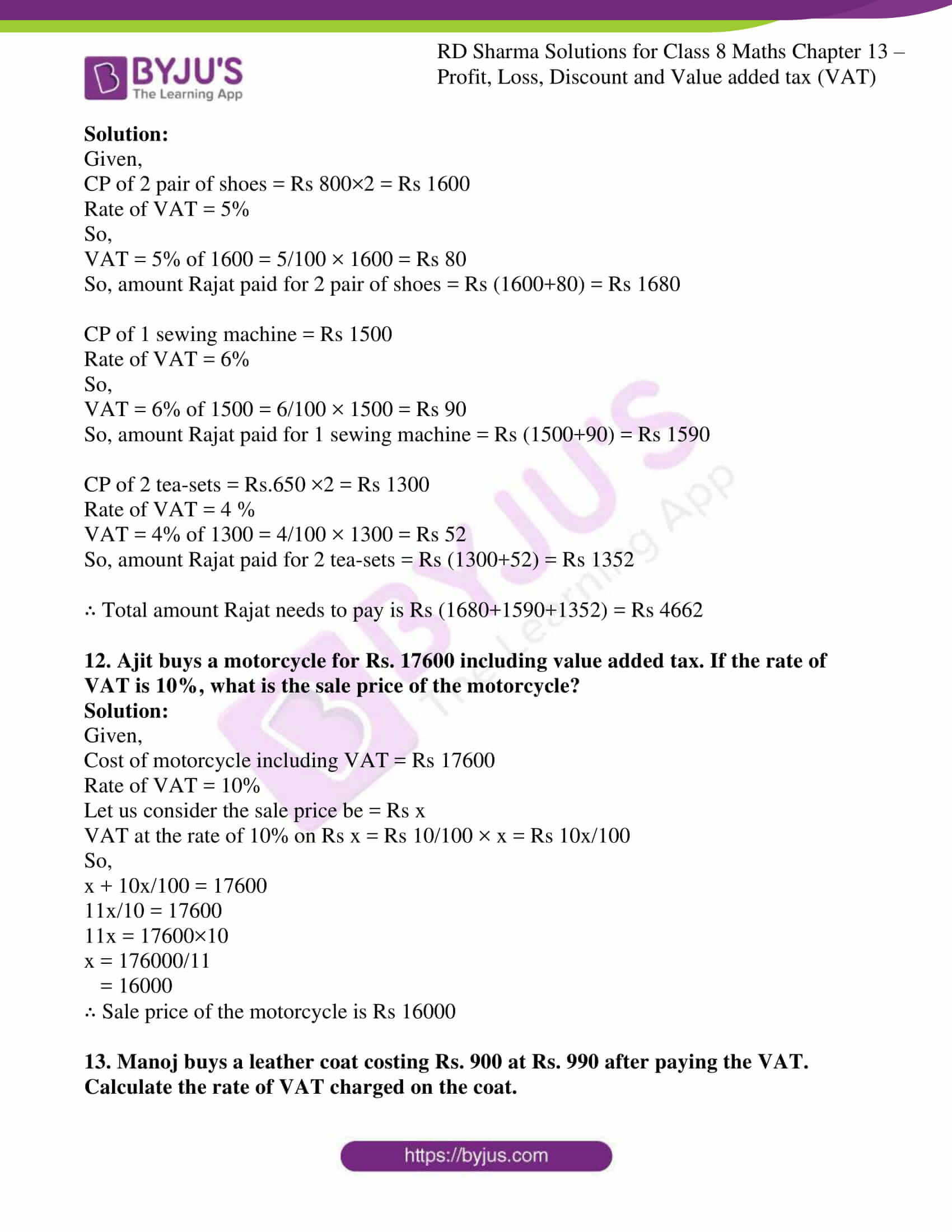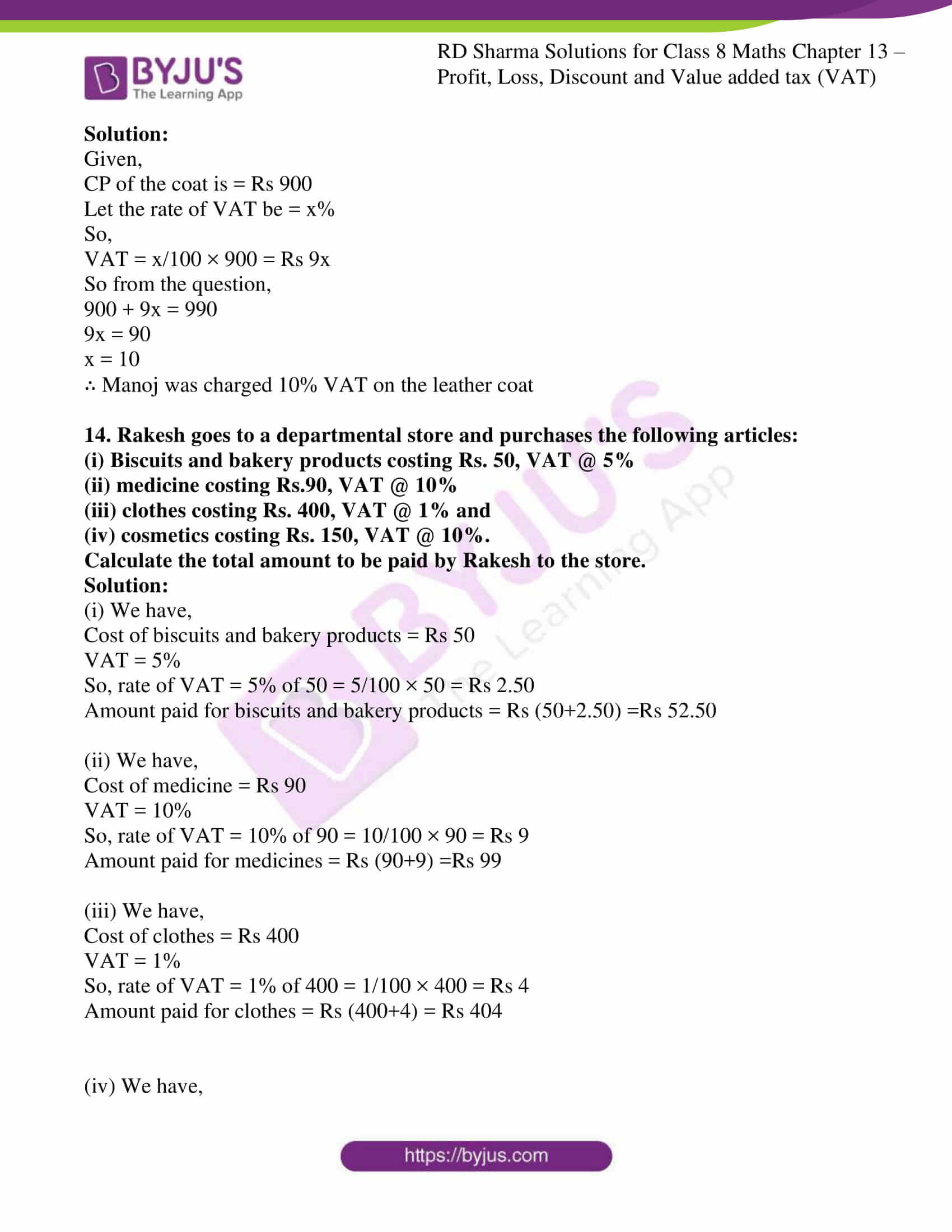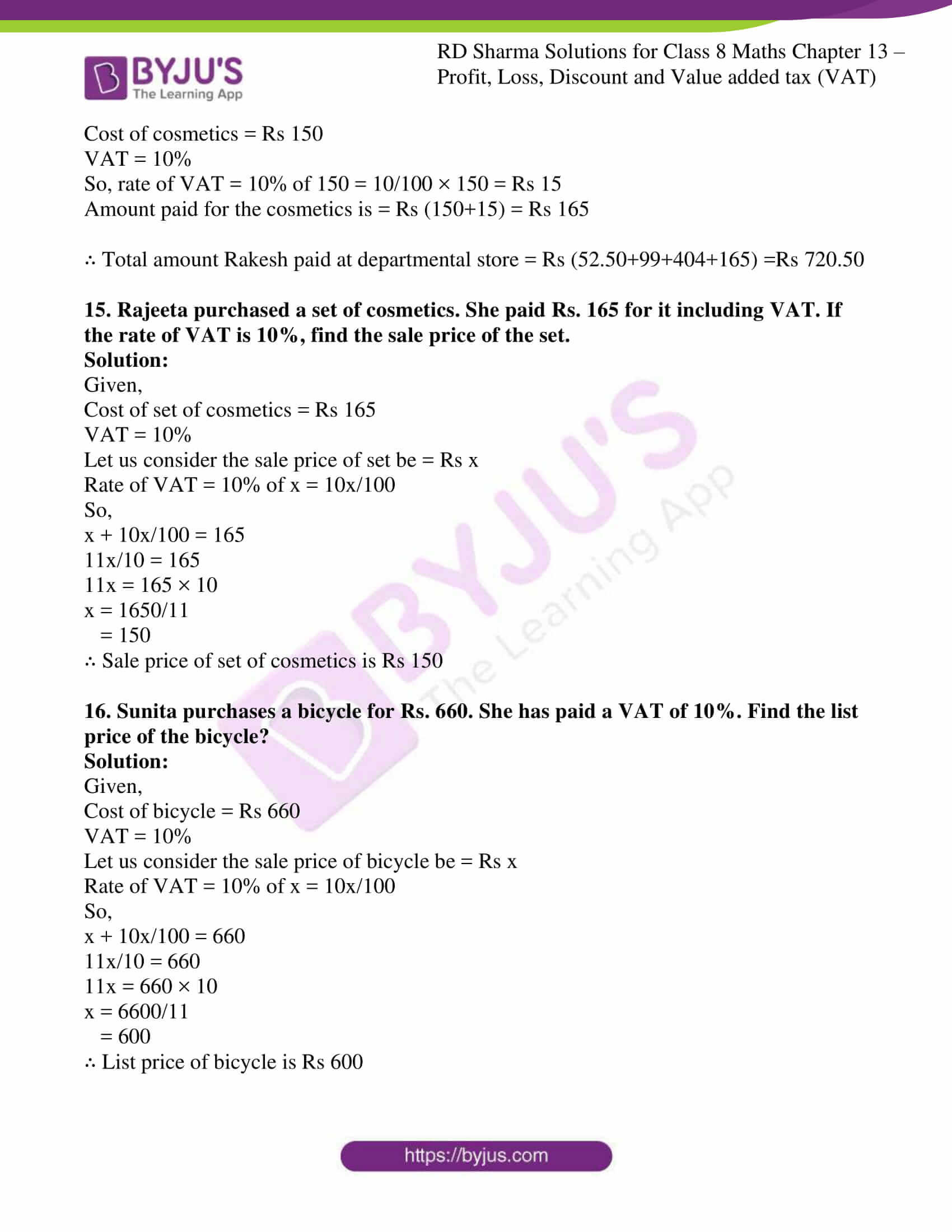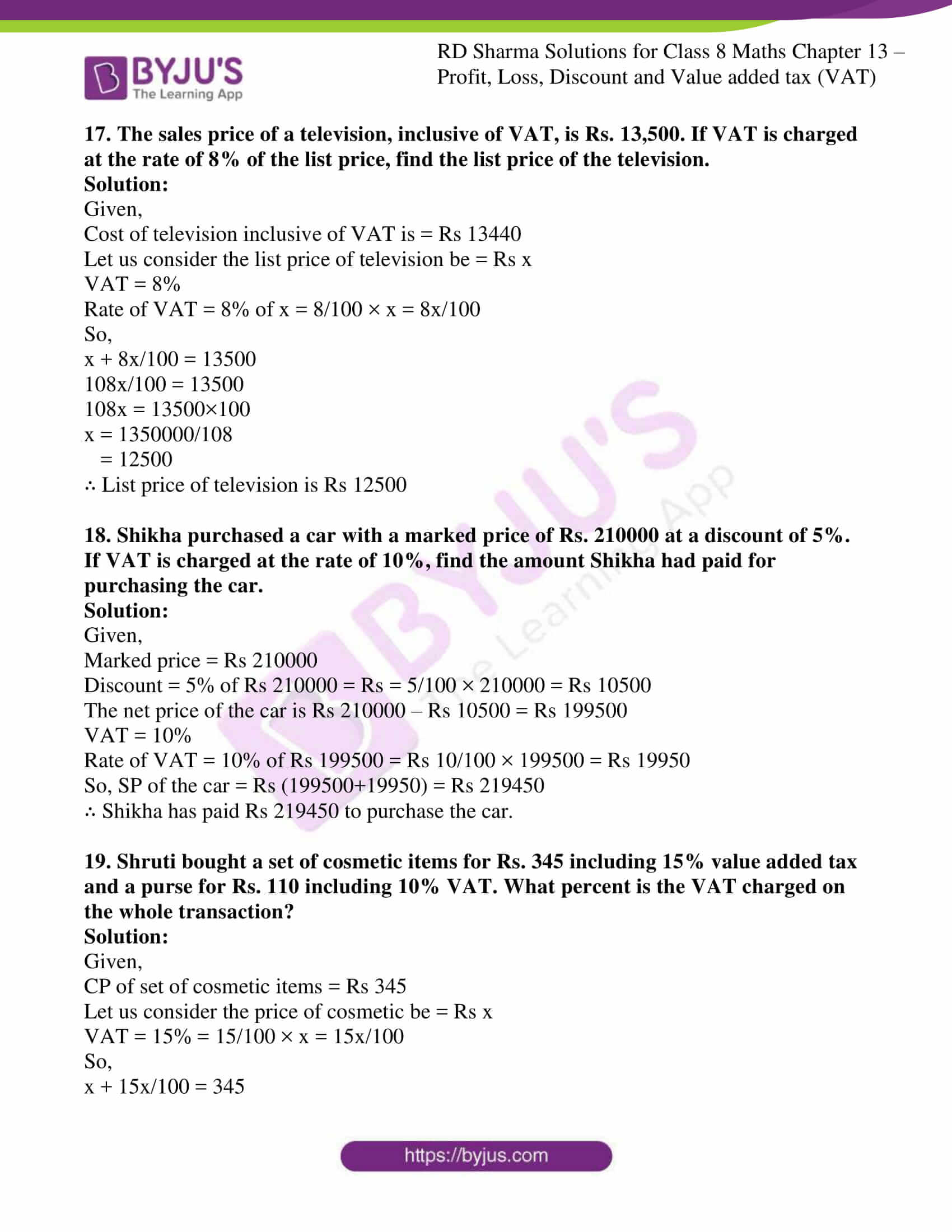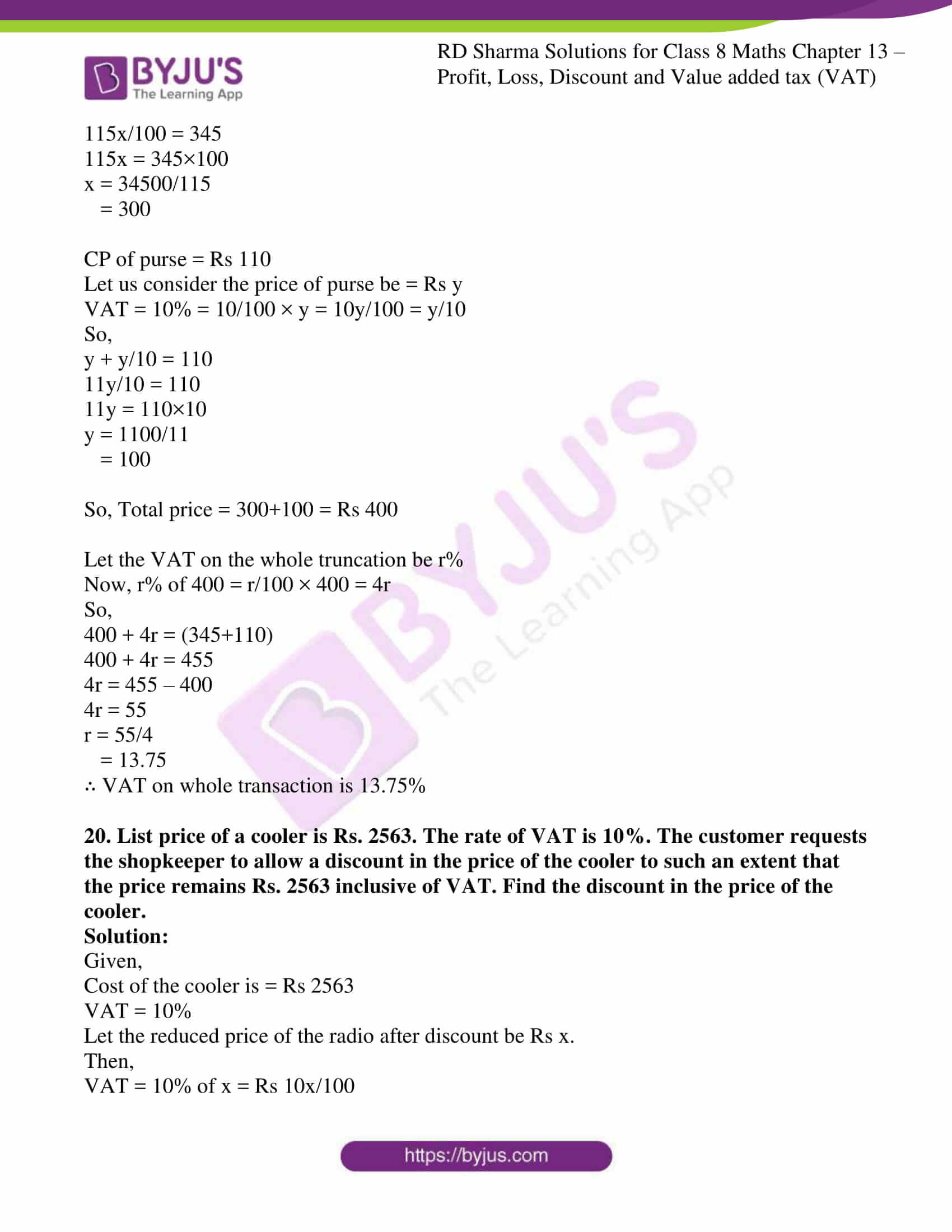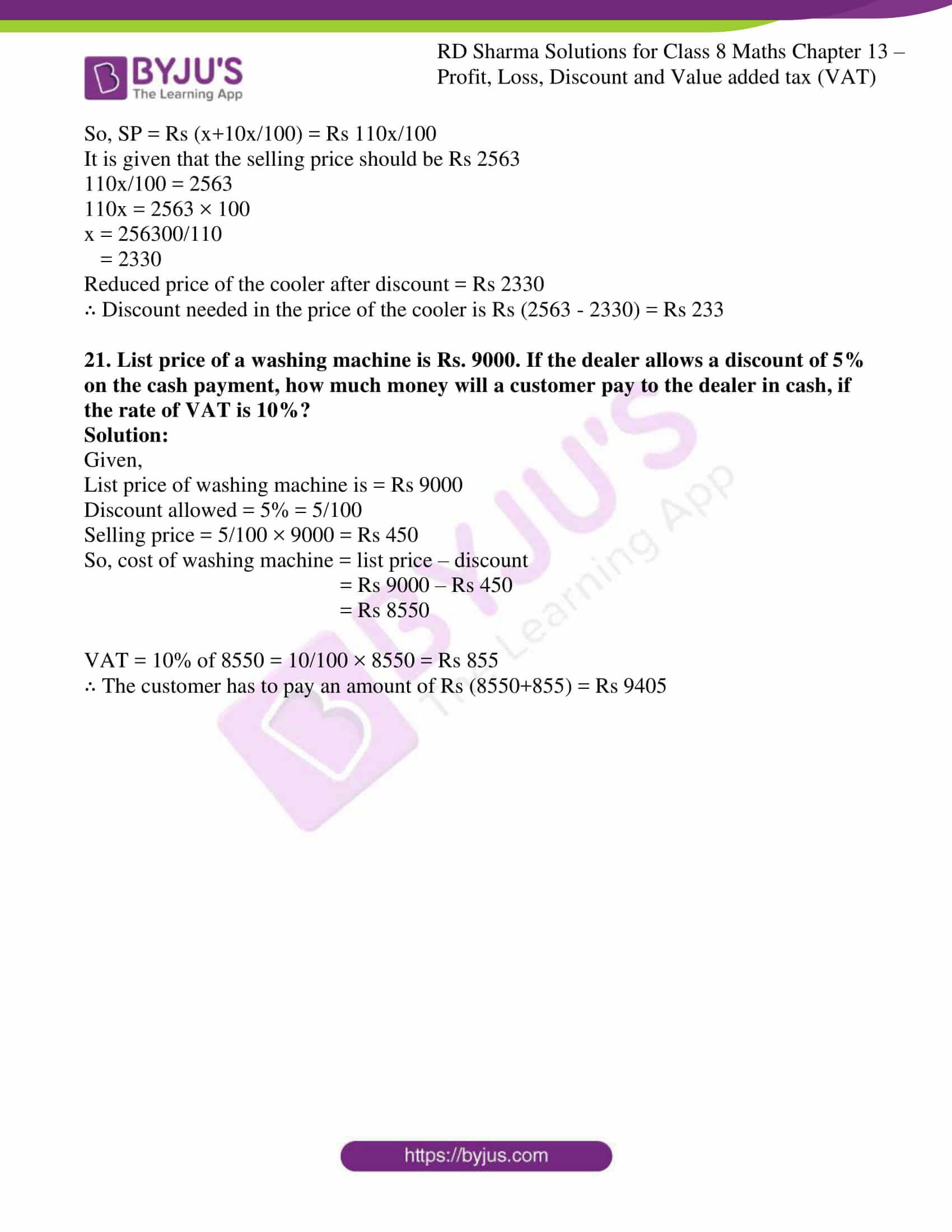### Access answers to Maths RD Sharma Solutions For Class 8 Chapter 13 Profit, Loss, Discount and Value Added Tax (VAT)

EXERCISE 13.1 PAGE NO: 13.11

1. A student buys a pen for Rs 90 and sells it for Rs 100. Find his gain and gain percent.

Solution:

We know that the cost price of pen = Rs 90

Selling price of pen = Rs 100

By using the formula,

Gain = selling price – cost price

= 100 – 90

= Rs 10

By using the formula,

Gain % = (gain/cost price) × 100

= (10/90) × 100

= 100/9

= $$11\frac{1}{9}$$ %

2. Rekha bought a saree for Rs 1240 and sold it for Rs 1147. Find her loss and loss percent.

Solution:

We know that the cost price of saree = Rs 1240

Selling price of saree = Rs 1147

By using the formula,

Loss = cost price – selling price

= 1240 – 1147

= Rs 93

By using the formula,

Loss % = (loss/cost price) × 100

= (93/1240) × 100

= 7.5 %

3. A boy buys 9 apples for Rs 9.60 and sells them at 11 for Rs 12. Find his gain or loss percent.

Solution:

We know that the cost price of 9 apples = Rs 9.60

Cost price of 1 apple = Rs 9.60/9

Selling price of 11 apple = Rs 12

Selling price of 1 apple = Rs 12/11

By using the formula,

Gain = selling price – cost price

= 12/11 – 9.60/9

= (108-105.60)/99

= Rs 2.40/99

By using the formula,

Gain % = (gain/cost price) × 100

= ((2.40/99)/(9.60/9)) × 100

= 25/11

= $$2\frac{3}{11}$$%

4. The cost price of 10 articles is equal to the selling price of 9 articles. Find the profit percent.

Solution:

We know that the cost price of 10 article = selling price of 9 article

Let us consider CP of 1 article as Rs X

Selling price of 9 article = 10X

Selling price of 1 article = 10x/9

Profit = 10x/9 – x

= x/9

Profit % = Gain % = (gain/cost price) × 100

= (x/9)/x × 100

= 100/9

= $$11\frac{1}{9}$$%

5. A retailer buys a radio for Rs 225. His overhead expenses are Rs 15. If he sells the radio for Rs 300, determine his profit percent.

Solution:

The cost price of a radio = Rs 225

Total cost = cost price + overhead expenses = 225+15 = Rs 240

Selling price of radio = Rs.300

By using the formula,

Gain = selling price – cost price

= 300 – 240 = Rs 60

By using the formula,

Gain % = (gain/cost price) × 100

= 60/240 × 100

= 25 %

6. A retailer buys a cooler for Rs 1200 and overhead expenses on it are Rs 40. If he sells the cooler for Rs 1550, determine his profit percent.

Solution:

We know the cost price of cooler = Rs 1200

Total cost = Rs 1200 + Rs 40 = Rs 1240

Selling price of cooler = Rs 1550

By using the formula,

Gain = selling price – cost price

= Rs 1550 – Rs1240

= Rs 310

By using the formula,

Gain % = (gain/cost price) × 100

= 310/1240 × 100

= 25%

7. A dealer buys a wristwatch for Rs 225 and spends Rs 15 on its repairs. If he sells the same for Rs 300, find his profit percent.

Solution:

We know the cost price of wrist watch = Rs 225

Cost of repairing = Rs 15

Total cost = Rs 225 + Rs 15 = Rs 240

Selling price of watch = Rs 300

By using the formula,

Gain = selling price – cost price

= Rs 300 – Rs 240

= Rs 60

By using the formula,

Gain % = (gain/cost price) × 100

= 60/240 × 100

= 25%

8. Ramesh bought two boxes for Rs 1300. He sold one box at a profit of 20% and the other box at a loss of 12%. If the selling price of both boxes is the same, find the cost price of each box.

Solution:

We know the cost price of two boxes = Rs 1300

So let us consider cost price of one box be Rs x

Cost price of other box = Rs 1300 – x

Selling price of first box = x + x × 20/100

= x + x/5

= Rs 6x/5

Selling price of second box = (1300 – x) – (1300 – x) × 12/100

= Rs (28600 – 22x)/25

By equating SP of first and second box we get,

6x/5 = (28600 – 22x)/25

150x = 28600 × 5 – 110x

150x + 110x = 28600 × 5

260x = 28600 × 5

x = (28600 × 5)/260

= 550

∴ Cost price of first box = Rs. 550

Cost price of second box = Rs1300 – Rs550 = Rs 750

9. If the selling price of 10 pens is equal to cost price of 14 pens, find the gain percent.

Solution:

Given that, Selling price of 10 pens = cost price of 14 pens

So, let the cost price of 1 pen be Rs x

Selling price of 10 pens = Rs 14x

Selling price of 1 pen =Rs 14x/10

By using the formula,

Gain = selling price – cost price

= 14x/10 – x

= 4x/10

By using the formula,

Gain % = (gain/cost price) × 100

= (4x/10)/x × 100

= 2/5 × 100

= 40%

10. If the selling price of 18 chairs be equal to selling price of 16 chairs, find the gain or loss percent.

Solution:

Given that, Cost price of 18 chairs = selling price of 16 chairs

So, let the cost price of 1 chair be Rs x

Selling price of 16 chairs =Rs 18x

Selling price of 1 chair = Rs 18x/16

By using the formula,

Gain = selling price – cost price

= 18x/16 – x

= 2x/16

= Rs x/8

By using the formula,

Gain % = (gain/cost price) × 100

= (x/8)/x × 100

= 25/2

= 12 ½ %

11. If the selling price of 18 oranges is equal to the cost price of 16 oranges, find the loss percent.

Solution:

Given that, Selling price of 18 oranges = cost price of 16 oranges

So, let the cost price of 1 orange be Rs x

Selling price of 18 oranges = Rs 16x

Selling price of 1 orange = Rs 16x/18

By using the formula,

Loss = cost price – selling price

= x – 16x/18

= 2x/18

= Rs x/9

By using the formula,

Loss % = (loss/cost price) × 100

= (x/9)/x × 100

= 100/9

= $$11\frac{1}{9}$$ %

12. Ravish sold his motorcycle to Vineet at a loss of 28%. Vineet spent Rs 1680 on its repairs and sold the motor cycle to Rahul for Rs 35910, thereby making a profit of 12.5%, find the cost price of the motor cycle for Ravish.

Solution:

Let us consider the cost price of motorcycle for Ravish be Rs x

Loss% for Ravish = 28%

Selling price for Ravish = x – x × 28/100 = (100x – 28x)/100 = 72x/100

= Rs 18x/25

Selling price for Ravish = cost price for Vineet = Rs 18x/25

Repair cost by Vineet = Rs 1680

Total cost price of the motorcycle for Vineet = Rs18x/25 + Rs 1680

Selling price for Vineet = Rs 35910

Profit = 35910 – (18x+42000)/25

= Rs (855750 – 18x)/25

Profit % = 12.5% (Given)

By using the formula,

Gain % = (gain/cost price) × 100

=> [(855750-18x)/25] / [(18x+42000)/25] × 100 = 12.5

=> [(855750-18x)/25] × [25/(18x+42000)] = 125/1000

=> (855750-18x) / (18x+42000) = 1/8

=> By cross multiplying we get

=> 8(855750-18x) = (18x+42000)

=> 6846000 – 144x = 18x + 42000

=> 6846000 – 42000 = 18x + 144x

=> 162x = 6804000

x = 6804000/162

= 42000

∴ Cost price of motorcycle for Ravish = Rs 42000

13. By selling a book for Rs 258, a bookseller gains 20%. For how much should he sell it to gain 30%?

Solution:

Given details are,

Selling price of book is = Rs 258

The man’s gain percent is = 20% of 100 = 20/100

So, let us consider the cost price of book be Rs x

By solving,

x + x×20/100 = 258

x + x/5 = 258

(5x+x)/5 = 258

By cross multiplying

6x= 5×258

x = 1290/6

= 215

Now, the cost price of book is = Rs 215

For a gain of 30% the man should sell the book at = 215 + 215×30/100

= 215 + 64.5

= 279.50

∴ To gain 30% the man should sell the book at Rs 279.50

14. A defective briefcase costing Rs 800 is being sold at a loss of 8%. If the price is further reduced by 5%, find its selling price.

Solution:

Given, cost price of the defective briefcase is = Rs. 800

The loss percent is = 8% of 100 = 8/100

Selling price of briefcase is = 800 – 800×8/100

= 800 – 64

= Rs 736

When the price is further reduced by 5% (Given) = 5% of 100 = 5/100

New selling price = 736 – 736×5/100

= 736 – 36.8

= Rs 699.2

∴ The selling price of the defective briefcase is Rs 699.2

15. By selling 90 ball pens for Rs 160 a person loses 20%. How many ball pens should be sold for Rs 96 so as to have profit of 20%?

Solution:

Given, selling price for 90 ball pens is = Rs 160

Selling price of 1 ball pen = Rs 160/90 = Rs 16/9

The loss percent is = 20% of 100 = 20/100

Let us consider the cost price of 1 pen be Rs x

By solving,

x – x×20/100 = 16/9

x – x/5 = 16/9

(5x-x)/5 = 16/9

4x/5 = 16/9

By cross multiplying

4x×9 = 16×5

36x = 80

x = 80/36

= Rs 20/9

Now, cost price of 1 ball pen = Rs 20/9

To get a profit of 20%…

Let us consider the number of pens be ‘x’

So, selling price of ‘x’ pens is = Rs 96

Selling price of 1 pen is = Rs 96/x

We know that,

Gain % = (gain/cost price) × 100

20% = [(96/x) – (20/9)] / (20/9) × 100

20/100 = [(96/x) – (20/9)] / (20/9) × 100

(20/100 × 200/9) + 200/90 = 96/x

4/9 + 200/90 = 96/x

(40+200)/90 = 96/x

240/90 = 96/x

24/9 = 96/x

By cross multiplying

24x = 96×9

x = 864/24

= 36

∴ 36 ball pens can be sold at a price of Rs 96

16. A man sells an article at a profit of 25%. If he had bought it at 20% less and sold it for Rs 36.75 less, he would have gained 30%. Find the cost price of the article.

Solution:

Let us consider the cost price of article be Rs x

The Profit percent is = 25% of 100 = 25/100

Selling price of article = x + x × 25/100

= x + x/4

= (4x+x)/4

= Rs 5x/4

If cost price of article is 20% less (given) = 20% of 100 = 20/100

Now, cost price is = x – x×20/100

= x – x/5

= (5x-x)/5

= Rs 4x/5

Now, selling price is = Rs5x/4 – 36.75

The Profit percent is = 30% of 100 = 30/100

He would have gained 30% selling at that price (Given)

We know that, Gain = SP – CP

= 5x/4 – 36.75 – 4x/5

= (25x – 16x)/20 – 36.75

= 9x/20 – 36.75

Gain % = (gain/cost price) × 100

30% = [{(5x/4) – 36.75} – (4x/5)] / (4x/5) × 100

30/100 = (9x/20 – 36.75) / (4x/5) × 100

x = 175

∴ Cost price of article is Rs 175

17. A dishonest shopkeeper professes to sell pulses at his cost price but uses a false weight of 950 gm for each kilogram. Find his gain percent.

Solution:

Let us consider the cost price of 1000gm pulses be Rs x

Selling price of 950 gm pulses is also = Rs x

Selling price of 1000 gm pulses = x/950 × 1000

So, Gain = SP – CP

Gain = 1000x/950 – x

= (1000x – 950x)/950

= 50x/950

Gain % = (gain/cost price) × 100

= (50x/950)/x × 100

= 50x/950x × 100

= 5/95 × 100

= 100/19

= $$5\frac{5}{19}$$%

∴ The Shopkeeper’s gain percent is $$5\frac{5}{19}$$%

18. A dealer bought two tables for Rs 3120. He sold one of them at loss of 15% and other at a gain of 36%. Then, he found that each table was sold for the same price. Find the cost price of each table.

Solution:

Given, the cost price of two tables is = Rs 3120

Let cost price of first table be = Rs x

Then, cost price of second table will be = Rs 3120 – x

We know that one is a gain and other is a loss.

Selling price of first table (gain) = x + x × 36/100

= x +9x/25

= (25x + 9x)/25

= Rs 34x/25

Selling price of second table (loss) = (3120 – x) × 85/100

= Rs (3120×85 – 85x)/100

So now, by equating both we get,

34x/25 = (3120×85 – 85x)/100

34x = (3120×85 – 85x)/4

34x × 4 = 3120×85 – 85x

136x + 85x = 3120×85

221x = 3120×85

x = (3120×85)/221

= 1200

∴ Cost price of first table (x) is = Rs 1200

Cost price of second table (3120 – x) = 3120 – 1200 = Rs 1920

19. Mariam bought two fans for Rs 3605. She sold one at a profit of 15% and the other at a loss of 9%. If Mariam obtained the same amount for each fan, find the cost price of each fan.

Solution:

Given, cost price of 2 fans is = Rs 3605

Let cost price of 1 fan be = Rs x

Then CP of other fan will be = Rs 3605 – x

We know that one is a gain and other is a loss.

Selling price of first fan (gain) = x + x×15/100

= x + x×3/20

= (20x+3x)/20

= Rs 23x/20

Selling price of second fan (loss) = (3605 – x) × 91/100

= Rs (3605×91 – 91x)/100

So now, by equating both we get,

23x/20 = (3605×91 – 91x)/100

23x = (3605×91 – 91x)/5

23x × 5 = 3605×91 – 91x

115x + 91x = 3605×91

206x = 3605×91

x = (3605×91)/206

= 1592.50

∴ Cost price of one fan (x) is = Rs 1592.50

Cost price of second fan (3605 – x) is = 3605 – 1592.50 = Rs 2012.50

20. Some toffees are bought at the rate of 11 for Rs 10 and the same number at the rate of 9 for Rs 10. If the whole lot is sold at one rupee per toffee, find the gain or loss percent on the whole transaction.

Solution:

Let the total number of toffees be ‘x’

Given, cost price of 11 toffees is = Rs 10

Cost price of 1 toffee is = Rs 10/11

Given, cost price of 9 toffees is = Rs 10

Cost price of 1 toffee is = Rs 10/9

When equating both the costs we get,

Cost price of two toffees = (10/11) + (10/9)

= (90 + 110)/99

= 200/99

Cost price of one toffee is = (Rs 200/99)/2 = Rs 200/198

We know that selling price of 1 toffee (Given) = Rs 1

Loss = CP – SP

= 200/198 – 1

= (200-198)/198

= 2/198

Loss% = (loss/cost price) × 100

= (2/198)/(200/198) × 100

= 2/198 × 198/200 × 100

= 2/200 × 100

= 2/2

= 1%

∴ It is 1% loss on the whole truncation.

21. A tricycle is sold at a gain of 16%. Had it been sold for Rs 100 more, the gain would have been 20%. Find the C.P. of the tricycle.

Solution:

Let us consider the cost price of tricycle be = Rs x

Selling price of the tricycle be = Rs x

Given, Gain% = 16% of 100 = 16/100

Selling price of tricycle = x + x×16/100

= (100x+16x)/100

= 116x/100

= 29x/25

Given, if selling price is Rs 100 more

New Selling price = 29x/25 + 100

Then, Gain% = 20%

By using the formula

Gain % = (gain/cost price) × 100 [by using Gain = SP – CP]

20 = [((29x/25)+100) – x] / x × 100

20x/100 = (29x + 2500 – 25x)/25

x/5 = (29x + 2500 – 25x)/25

5x = 4x + 2500

x = 2500

∴ Cost price of tricycle is Rs 2500

22. Shabana bought 16 dozen ball bens and sold them at a loss equal to S.P. of 8 ball pens. Find
(i) her loss percent
(ii) S.P. of 1 dozen ball pens, if she purchased these 16 dozen ball pens for Rs 576.

Solution:

Given, number of ball pens bought by Shabana is = 16 dozen = 16×12 = 192 pens

So, let’s consider the cost price of each pen as Rs x

CP of 8 pens = Rs 8x

Let SP of one pen be = Rs x

So, SP of 192 pens = 192x

Given, loss of 192 pens = SP of 8 ball pens

So, loss = 8SP

192x = (192+8) SP

SP = 192x/200

Loss = CP – SP

= x – 192x/200

(i) Loss% = (loss/CP) × 100

= (x – 192x/200)/x × 100

= (200x-192x)/200x × 100

= 8/2

= 4%

(ii) Given, CP of 16 dozen pens = Rs 576

192x = 576

x = 576/192

We know that SP of 1 pen = 192x/200

SP of dozen pens = 12 × 192x/200

= 12 × 192/200 × 576/192

= 12 × 576/200

= 34.56

∴ Loss% = 4% and SP of 1 dozen pens is Rs 34.56

23. The difference between two selling prices of a shirt at profits of 4% and 5% is Rs 6. Find
(i) C.P. of the shirt
(ii) The two selling prices of the shirt

Solution:

(i) Let the CP of shirt be = Rs x

Profit (4%) = 4/100 of CP

= 4/100 × x

= 4x/100

Selling Price = C.P + Profit

= x + 4x/100

= (100x + 4x)/100

= 104x/100

(ii) Let the CP of shirt be = Rs x

Profit (5%) = 5/100 of CP

= 5/100 × x

= 5x/100

Selling Price = C.P + Profit

= x + 5x/100

= (100x + 5x)/100

= 105x/100

Given that, the difference between the two selling price is Rs 6

So, 105x/100 – 104x/100 = 6

(105x-104x)/100 = 6

x/100 = 6

x = 600

∴ Now, C.P of the shirt is = Rs 600

Selling Price of one shirt = 104x/100 = (104×600)/100 = Rs 624

Selling Price of other shirt = 105x/100 = (105×600)/100 = Rs 630

24. Toshiba bought 100 hens for Rs 8000 and sold 20 of these at a gain of 5%. At what gain percent she must sell the remaining hens so as to gain 20% on the whole?

Solution:

Given, Total hens = 100

Remaining hens = 100-20 = 80 hens

Toshiba bought 100 hens for = Rs 8000

1 hen cost is = 8000/100 = Rs 80

20hens cost = 20 × 80 = Rs 1600

Given, Gain = 5%

SP = 105/100 × 1600

= Rs 1680

CP for 80 hens = 80 × 80 = Rs 6400

SP of 80 hens = Rs (1600 + 6400-80) = Rs 7920

Gain on 80 hens = SP of 80 hens – CP of 80 hens

= 7920 – 6400

= Rs 1520

Gain % = (gain/cost price) × 100

Gain% on 80 hens = (1520/6400) × 100

= 23.75%

∴ Toshiba require 23.75% gain on the remaining hens (80hens).

EXERCISE 13.2 PAGES NO: 13.26

1. Find the S.P. if
(i) M.P. = Rs 1300 and Discount = 10%
(ii) M.P. = Rs 500 and Discount = 15%

Solution:

(i) Given,

M.P. = 1300

Discount = 10%

By using the formulas

SP = Marked price (MP) – Discount

Discount = (MP × Discount %)/100

Discount% = (Discount)/M.P. × 100

By using,

Discount = (MP × Discount %)/100

= (1300×10)/100

= Rs 130

SP = MP – Discount

= (1300 – 130) = Rs 1170

(ii) Given,

M.P. = 500

Discount = 15%

By using,

Discount = (MP × Discount %)/100

= (500×15)/100

= Rs 75

SP = MP – Discount

= (500 – 75) = Rs 425

2. Find the M.P. if
(i) S.P. = Rs 1222 and Discount = 6%
(ii) S.P. = Rs 495 and Discount = 1%

Solution:

(i) Given,

SP = Rs 1222

Discount = 6%

By using the formula

MP = (100 × SP) / (100 – Discount %)

= (100 × 1222) / (100 – 6)

= 122200/94

= Rs 1300

(ii) Given,

SP = Rs 495

Discount = 1%

By using the formula

MP = (100 × SP) / (100 – Discount %)

= (100 × 495) / (100 – 1)

= 49500/99

= Rs 500

3. Find the discount in percent when
(i) M.P. = Rs. 900 and S.P. = Rs. 873
(ii) M.P. = Rs. 500 and S.P. = Rs. 425

Solution:

(i) Given,

MP = Rs 900

SP = Rs 873

By using the formula

Discount% = (MP – SP)/MP × 100

= (900-873)/900 × 100

= 27/900 × 100

= 3%

(ii) Given,

MP = Rs 500

SP = Rs 425

By using the formula

Discount% = (MP – SP)/MP × 100

= (500-425)/500 × 100

= 75/500 × 100

= 15%

4. A shop selling sewing machines offers 3% discount on all cash purchases. What cash amount does a customer pay for a sewing machine the price of which is marked as Rs 650.

Solution:

Given,

MP = Rs 650

Discount = 3%

So, 3% of MP = 3/100 × 650

= Rs 19.5

MP = MP – discount

= 650 – 19.5

= Rs 630.5

∴ Customer has to pay Rs 630.50

5. The marked price of a ceiling fan is Rs 720. During off season, it is sold for Rs. 684. Determine the discount percent.

Solution:

Given,

MP = Rs 720

SP = Rs 684

By using the formula,

Discount = M.P. – S.P.

= 720 – 684 = Rs 36

Discount% = (Discount/MP) × 100

= 36/720 × 100

= 5%

∴ Discount% is 5%

6. On the eve of Gandhi Jayanti a saree is sold for Rs. 720 after allowing 20% discount. What is its marked price?

Solution:

Given,

SP of the saree = Rs 720

Discount = 20%

By using the formula

MP = (100 × SP) / (100 – Discount %)

= (100 × 720) / (100 – 20)

= 72000/80

= Rs 900

∴ Marked Price = Rs 900

7. After allowing a discount of 7½ % on the marked price, an article is sold for Rs. 555. Find its marked price.

Solution:

Given,

SP of the article = Rs 555

Discount = 7½ % = 15/2%

By using the formula

MP = (100 × SP) / (100 – Discount %)

= (100 × 555) / (100 – (15/2))

= (100 × 555) / ((200 – 15)/2)

= (100 × 555) / (92.5)

= 55500/92.5

= Rs 600

∴ Marked Price = Rs 600

8. A shopkeeper allows his customers 10% off on the marked price of goods and still gets a profit of 25%. What is the actual cost to him of an article marked Rs. 250?

Solution:

Given, 10% off on marked price

M.P. = 250

Discount = 10%

By using,

Discount = (MP × Discount %)/100

= (250×10)/100

= Rs 25

SP = MP – Discount

= (250 – 25) = Rs 225

And 25% profit he gets additionally,

So, by using the formula,

CP = 100 / (100 + Gain %) × SP

= 100 / (100 + 25) × 225

= 100/125 × 225

= 180

∴ Actual cost of the article is Rs 180

9. A shopkeeper allows 20% off on the marked price of goods and still gets a profit of 25%. What is the actual cost to him of an article marked Rs. 500?

Solution:

Given, 20% off on marked price

MP = 500

Discount = 20%

Discount = (MP × Discount %)/100

= (500×20)/100

= Rs 100

SP = MP – Discount

= (500 – 100) = Rs 400

And 25% profit he gets additionally,

So, by using the formula,

CP = 100 / (100 + Gain %) × SP

= 100 / (100 + 25) × 400

= 100/125 × 400

= 320

∴ Actual cost of the article is Rs 320

10. A tradesman marks his goods at such a price that after allowing a discount of 15%, he makes a profit of 20%. What is the marked price of an article whose cost price is Rs. 170?

Solution:

Given,

CP of the article = Rs 170

Profit = 20%

So, by using the formula,

Selling price = (100 + Gain %)/100 × CP

= (100 + 20)/100 × 170

= 120/100 × 170

= 204

SP = Rs 204

Discount = 15%

By using the formula

MP = (100 × SP) / (100 – Discount %)

= (100 × 204) / (100 – 15)

= (100 × 204) / 85

= 20400/85

= Rs 240

∴ Marked Price = Rs 240

11. A shopkeeper marks his goods in such a way that after allowing a discount of 25% on the marked price, he still makes a profit of 50%. Find the ratio of the C.P. to the M.P.

Solution:

Given,

Discount = 25%

Discount = (MP × Discount %)/100

= (MP×25)/100

= Rs 25MP/100

SP = MP – Discount

= (MP – 25MP/100)

= (100MP – 25MP)/100

= 75MP/100

We know that the given profit = 50%

Selling price = (100 + Gain %)/100 × CP

= (100 + 50)/100 × CP

= 150/100 × CP

= 150CP/100

By equating both SP we get,

75MP/100 = 150CP/100

75MP/150CP = 100/100

75MP/150CP = 1

(By cross multiplying)

CP/MP = 75/150

= 1/2

∴ The ratio CP to MP = 1:2

12. A cycle dealer offers a discount of 10% and still makes a profit of 26%. What is the actual cost to him of a cycle whose marked price is Rs. 840?

Solution:

Given,

Marked price (MP) on cycle = Rs 840

Discount = 10%

Discount = (MP × Discount %)/100

= (840×10)/100

= Rs 84

SP = MP – Discount

= 840 – 84

= Rs 756

Given, he makes a profit of 26% additionally

So, by using the formula,

CP = 100 / (100 + Gain %) × SP

= 100 / (100 + 26) × 756

= 100/126 × 756

= 600

∴ Actual cost of the cycle is Rs 600

13. A shopkeeper allows 23% commission in his advertised price and still makes a profit of 10%. If he gains Rs. 56 on one item, find his advertised price.

Solution:

Let us consider the advertised price be = Rs x

And the commission on the advertised price = 23% = Rs 23x/100

Selling price = advertised price – commission

= x – 23x/100

= (100x – 23x)/100

= Rs 77x/100 ……(equation 1)

Given gain = Rs 56

Profit percent = 10%

So, by using the formula,

Gain% = (gain/CP) × 100

10 = (56/CP) × 100

10/100 = 56/CP

CP = 560

Gain = SP – CP

SP = 560 + 56 = Rs 616

From the above equation 1 we get,

77x/100 = 616

x = (616 × 100)/77

= 800

∴ advertised price is Rs 800

14. A shopkeeper marked his goods at 40% above the cost price but allows a discount of 5% for cash payment to his customers. What actual profit does he make, if he receive Rs. 1064 after paying the discount?

Solution:

Given,

Shopkeeper marks his goods at 40% above the cost price.

Let the cost price be ‘x’

Marked price is 140x/100 (40 more than 100 if CP is 100)

Discount on marked price is 5%

Discount = (MP × Discount %)/100

= (140x/100×5)/100

= (7x/100)

SP = MP – Discount

= 140x/100 – 7x/100

= (140x-7x)/100

= Rs 133x/100

Given SP = Rs 1064

Equating both the SP we get,

1064 = 133x/100

133x = 1064×100

x = (1064×100)/133

= 800

Now, the cost price = Rs 800

SP = Rs 1064

Profit = SP – CP

= 1064 – 800

= 264

∴ The actual profit is Rs 264

15. By selling a pair of earrings at a discount of 25% on the marked price, a jeweller makes a profit of 16%. If the profit is Rs. 48, what is the cost price? What is the marked price and the price at which the pair was eventually bought?

Solution:

Given,

Earrings are bought at 25% discount

Profit percent of seller = 16%

Gain = Rs 48

So, by using the formula,

Gain% = (gain/CP) × 100

16 = (48/CP) × 100

16/100 = 48/CP

CP = (48×100)/16

= Rs 300

Now,

CP = Rs 300

Cost price of the earrings = Rs 300

Profit = Rs 48

Profit = SP – CP

SP = Profit + CP

= 48 + 300

= 348

By using the formula

MP = (100 × SP) / (100 – Discount %)

= (100 × 348) / (100 – 25)

= (100 × 348) / 75

= 34800/75

= Rs 464

∴ Marked Price is Rs 464, CP is 300 and Final selling price is 348

16. A publisher gives 32% discount on the printed price of a book to booksellers. What does a book seller pay for a book whose printed price is Rs. 275?

Solution:

Given,

Printed price (MP) = Rs 275

Discount = 32%

Discount = (MP × Discount %)/100

= (275×32)/100

= 88

SP = MP – Discount

= 275 – 88

= Rs 187

∴ The book seller pays Rs 187

17. After allowing a discount of 20% on the marked price of a lamp, a trader loses 10%. By what percentage is the marked price above the cost price?

Solution:

Given,

Let the cost price of the lamp be = Rs x

Then, SP = (100 – loss%)/100 × CP

= (100 – 10)/100 × x

= 90x/100

Now,

SP = Rs 90x/100 and Discount = 20%

By using the formula,

MP = (100×SP) / (100 – Discount%)

= (100×90x/100) / (100 – 20)

= 90x/80

= 9x/8

Required difference = MP – CP

= Rs (9x/8 – x)

= (9x-8x)/8

= Rs x/8

Discount% = (x/8)/x × 100

= 100/8

= 12.5%

∴ The trader must mark his goods 12.5% above the cost price.

18. The list price of a table fan is Rs. 480 and it is available to a retailer at 25% discount. For how much should a retailer sell it to gain 15%?

Solution:

Given,

List price of table fan (MP) is = Rs 480

Retailer buys it at discount of = 25%

Cost price for the retailer is (75/100) × 480

So, CP = (75/100) × 480

= Rs 360

Now, the retailer sells the fan to get 15%

Gain% = (gain)/CP × 100

15% = (SP-CP)/CP × 100

SP = 115/100 CP

= 115/100 × 360

= 414

∴ The retailer should sell the fan at Rs 414 to get a gain of 15%

19. Rohit buys items at 25% discount on the marked price. He sells it for Rs. 660, making a profit of 10%. What is the marked price of the item?

Solution: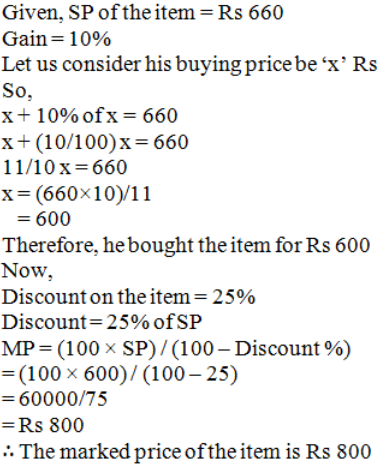20. A cycle merchant allows 20% discount on the marked price of the cycles and still makes a profit of 20%. If he gains Rs. 360 over the sale of one cycle, find the marked price of the cycle.

Solution:

Given,

Profit% = 20%

Gain over 1 cycle = Rs 360

Gain% = (gain/CP) × 100

20 = 360/CP × 100

20/100 = 360/CP

CP = Rs 1800

By using the formula,

SP = (100 + gain%)/100 × CP

= (100 + 20)/100 × 1800

= 120/100 × 1800

= Rs 2160

Given, Discount = 20%

MP = (100 × SP) / (100 – Discount %)

= (100 × 2160) / (100 – 20)

= 216000/80

= Rs 2700

∴ The marked price of the cycle is Rs 2700

21. Jyoti and Meena run a ready-made garment shop. They mark the garments at such a price that even after allowing a discount of 12.5%, they make a profit of 10%. Find the marked price of a suit which costs them Rs. 1470.

Solution:

Given,

CP of suit = Rs 1470

Gain = 10%

By using the formula,

SP = (100 + gain%)/100 × CP

= (100 + 10)/100 × 1470

= 110/100 × 1470

= Rs 1617

Given, Discount = 12.5%

So, MP = (100 × SP) / (100 – Discount %)

= (100 × 1617) / (100 – 12.5)

= 161700/87.5

= Rs 1848

∴ The marked price of the suit is Rs 1848

22. What price should Aslam mark on a pair of shoes which costs him Rs. 1200 so as to gain 12% after allowing a discount of 16%?

Solution:

Given,

CP of pair of shoes = Rs 1200

Gain = 12%

By using the formula,

SP = (100 + gain %)/100 × CP

= (100 + 12)/100 × 1200

= 112/100 × 1200

= Rs 1344

Given, Discount = 16%

MP = (100 × SP) / (100 – Discount %)

= (100 × 1344) / (100 – 16)

= 134400/84

= Rs 1600

∴ Aslam should mark pair of the shoes at Rs 1600

23. Jasmine allows 4% discount on the marked price of her goods and still earns a profit of 20%. What is the cost price of a shirt for her marked at Rs. 850?

Solution:

Given,

Gain = 20%

MP of the shirt = Rs 850

Discount = 4%

Discount allowed on the marked price of goods = 4/100 × 850

= Rs 34

So, SP of the shirt = (850 – 34) = Rs 816

By using the formula,

CP = 100/(100 + gain%) × SP

= 100/(100 + 20) × 816

= 100/120 × 816

= Rs 680

∴ Cost price of a shirt is Rs 680

24. A shopkeeper offers 10% off-season discount to the customers and still makes a profit of 26%. What is the cost price for the shopkeeper on a pair of shoes marked at Rs. 1120?

Solution:

Given,

MP of pair of shoes = Rs 1120

Profit = 26%

Discount = 10%

Discount allowed = 10/100 × 1120

= Rs 112

So, SP of the shoes = (1120 – 112) = Rs 1008

By using the formula,

CP = 100/(100 + gain%) × SP

= 100/(100 + 26) × 1008

= 100/126 × 1008

= Rs 800

∴ Cost price of a pair shoes is Rs 800

25. A lady shopkeeper allows her customers 10% discount on the marked price of the goods and still gets a profit of 25%. What is the cost price of a fan for her marked at Rs. 1250?

Solution:

Given,

MP of the fan = Rs 1250

Profit = 25%

Discount = 10%

Discount allowed = 10/100 × 1250

= Rs 125

So, SP of the shoes = (1250 – 125) = Rs 1125

By using the formula,

CP = 100/(100 + gain%) × SP

= 100/(100 + 25) × 1125

= 100/125 × 1125

= Rs 900

∴ Cost price of the fan is Rs 900

EXERCISE 13.3 PAGES NO: 13.32

1. The list price of a refrigerator is Rs. 9700. If a value added tax of 6% is to be charged on it, how much one has to pay to buy the refrigerator?

Solution:

Given,

The list price of a refrigerator is = Rs 9700

VAT = 6%

Rate of VAT = 6% of Rs 9700

= 6/100 × 9700

= Rs 582

∴ The total amount one has to pay to buy refrigerator is Rs 9700 + Rs 582 = Rs 10282

2. Vikram bought a watch for Rs. 825. If this amount includes 10% VAT on the list prices. What was the list price of the watch?

Given,

Vikram bought watch for = Rs 825

VAT = 10%

Let the list price of the watch be = Rs x

VAT at the rate of 10% on Rs x = Rs 10/100 × x = Rs x/10

So, the list price of the watch = Rs (x + x/10) = Rs 11x/10

It is given that watch is bought at the price of Rs 825

∴ 11x/10 = 825

x = (825×10)/11

= 750

∴ List price of the watch is Rs 750

3. Aman bought a shirt for Rs. 374.50 which includes 7% VAT. Find the list price of the shirt.

Solution:

Given,

Aman bought a shirt for = Rs 374.50

VAT = 7%

Let the list price of the shirt be = Rs x

VAT at the rate of 7% on Rs x = Rs 7/100 × x = Rs 7x/100

So, the list price of the shirt = Rs (x + 7x/100) = Rs 107x/100

It is given that shirt is bought at the price of Rs 374.50

∴ 107x/100 = 374.50

x = (374.50×100)/107

= 350

∴ List price of the shirt is Rs 350

4. Rani purchases a pair of shoes whose sale price is Rs. 175. If she pays VAT at the rate of 7%, how much amount does she pay as VAT? Also, find the net value of the pair of shoes.

Solution:

Given,

Sale price of shoes = Rs.175

VAT = 7%

VAT at the rate 7% of 175 = 7/100 × 175 = Rs 12.25

∴ Net value of pair of shoes = sale price +VAT

= 175 + 12.25 = Rs 187.25

5. Swarna paid Rs. 20 as VAT on a pair of shoes worth Rs. 250. Find the rate of VAT.

Solution:

Given,

List price of shoes = Rs.250

Let VAT be = x%

So, VAT at the rate x% of 250 = 20 (Given)

x = (20×100)/250 = 8%

∴ VAT = 8%

6. Sarita buys goods worth Rs. 5500. She gets a rebate of 5% on it. After getting the rebate if VAT at the rate of 5% is charged, find the amount she will have to pay for the goods.

Solution:

Given,

Price of goods is = Rs 5500

Discount = 5%

VAT = 5% of selling price

So,

Selling price = 95/100 × 5500 = Rs 5225

And,

VAT = 5/100 × 5225 = Rs 261.25

∴ Sarita has to pay an amount of Rs (5225 + 261.25) = Rs 5486.25 for the goods

7. The cost of furniture inclusive of VAT is Rs. 7150. If the rate of VAT is 10%, find the original cost of the furniture.

Solution:

Given,

Cost of furniture inclusive of VAT is = Rs 7150

VAT = 10%

Let us consider the original cost of furniture be = Rs x

VAT at the rate of 10% on Rs x = Rs 10/100 × x = Rs 10x/100

So,

x + 10x/100 = 7150

(100x+10x)/100 = 7150

110x/100 =7150

11x/10 = 7150

11x = 7150 × 10

x = 71500/11

= 6500

∴ Original cost of the furniture is Rs 6500

8. A refrigerator is available for Rs. 13750 including VAT. If the rate of VAT is 10%, find the original cost of the refrigerator.

Solution:

Given,

Cost of refrigerator inclusive of VAT is = Rs 13750

VAT = 10%

Let us consider the original cost of refrigerator be = Rs x

VAT at the rate of 10% on Rs x = Rs 10/100 × x = Rs 10x/100

So,

x + 10x/100 = 13750

(100x+10x)/100 = 13750

110x/100 = 13750

11x/10 = 13750

11x = 13750 × 10

x = 137500/11

= 12500

∴ Original cost of the refrigerator is Rs 12500

9. A colour T.V. is available for Rs. 13440 inclusive of VAT. If the original cost of TV is Rs. 12000, find the rate of VAT.

Solution:

Given,

Cost of TV inclusive of VAT is = Rs 13440

Original cost of the TV is = Rs 12000

So, let us consider the rate of VAT = x%

VAT at the rate of x% on Rs 12000 = Rs x/100 × 12000 = Rs 120x

So,

12000 + 120x = 13440

120x = 13440-12000

120x = 1440

x = 1440/120

= 12

∴ Rate of VAT = 12%

10. Reena goes to a shop to buy a radio, costing Rs. 2568. The rate of VAT is 7%. She tells the shopkeeper to reduce the price of the radio such that she has to pay Rs. 2568, inclusive of VAT. Find the reduction needed in the price of radio.

Solution:

Given,

Cost of the radio is = Rs 2568

VAT = 7%

Let the reduced price of the radio after discount be Rs x.

Then,

VAT = 7% of x = Rs 7x/100

So, SP = Rs (x+7x/100) = Rs 107x/100

It is given that the selling price should be Rs 2568

107x/100 = 2568

107x = 2568 × 100

x = 256800/107

= 2400

Reduced price of the radio after discount = Rs 2400

∴ Reduction needed in the price of the radio is Rs (2568 – 2400) = Rs 168

11. Rajat goes to a departmental store and buys the following articles:

 Item Price per item Rate of VAT 2 Pairs of shoes 1 sewing machine 2 tea – sets Rs 800 Rs 1500 Rs 650 5% 6% 4%

Calculate the total amount he has to pay to the store.

Solution:

Given,

CP of 2 pair of shoes = Rs 800×2 = Rs 1600

Rate of VAT = 5%

So,

VAT = 5% of 1600 = 5/100 × 1600 = Rs 80

So, amount Rajat paid for 2 pair of shoes = Rs (1600+80) = Rs 1680

CP of 1 sewing machine = Rs 1500

Rate of VAT = 6%

So,

VAT = 6% of 1500 = 6/100 × 1500 = Rs 90

So, amount Rajat paid for 1 sewing machine = Rs (1500+90) = Rs 1590

CP of 2 tea-sets = Rs.650 ×2 = Rs 1300

Rate of VAT = 4 %

VAT = 4% of 1300 = 4/100 × 1300 = Rs 52

So, amount Rajat paid for 2 tea-sets = Rs (1300+52) = Rs 1352

∴ Total amount Rajat needs to pay is Rs (1680+1590+1352) = Rs 4662

12. Ajit buys a motorcycle for Rs. 17600 including value added tax. If the rate of VAT is 10%, what is the sale price of the motorcycle?

Solution:

Given,

Cost of motorcycle including VAT = Rs 17600

Rate of VAT = 10%

Let us consider the sale price be = Rs x

VAT at the rate of 10% on Rs x = Rs 10/100 × x = Rs 10x/100

So,

x + 10x/100 = 17600

11x/10 = 17600

11x = 17600×10

x = 176000/11

= 16000

∴ Sale price of the motorcycle is Rs 16000

13. Manoj buys a leather coat costing Rs. 900 at Rs. 990 after paying the VAT. Calculate the rate of VAT charged on the coat.

Solution:

Given,

CP of the coat is = Rs 900

Let the rate of VAT be = x%

So,

VAT = x/100 × 900 = Rs 9x

So from the question,

900 + 9x = 990

9x = 90

x = 10

∴ Manoj was charged 10% VAT on the leather coat

14. Rakesh goes to a departmental store and purchases the following articles:

(i) Biscuits and bakery products costing Rs. 50, VAT @ 5%
(ii) medicine costing Rs.90, VAT @ 10%
(iii) clothes costing Rs. 400, VAT @ 1% and
(iv) cosmetics costing Rs. 150, VAT @ 10%.
Calculate the total amount to be paid by Rakesh to the store.

Solution:

(i) We have,

Cost of biscuits and bakery products = Rs 50

VAT = 5%

So, rate of VAT = 5% of 50 = 5/100 × 50 = Rs 2.50

Amount paid for biscuits and bakery products = Rs (50+2.50) =Rs 52.50

(ii) We have,

Cost of medicine = Rs 90

VAT = 10%

So, rate of VAT = 10% of 90 = 10/100 × 90 = Rs 9

Amount paid for medicines = Rs (90+9) =Rs 99

(iii) We have,

Cost of clothes = Rs 400

VAT = 1%

So, rate of VAT = 1% of 400 = 1/100 × 400 = Rs 4

Amount paid for clothes = Rs (400+4) = Rs 404

(iv) We have,

Cost of cosmetics = Rs 150

VAT = 10%

So, rate of VAT = 10% of 150 = 10/100 × 150 = Rs 15

Amount paid for the cosmetics is = Rs (150+15) = Rs 165

∴ Total amount Rakesh paid at departmental store = Rs (52.50+99+404+165) =Rs 720.50

15. Rajeeta purchased a set of cosmetics. She paid Rs. 165 for it including VAT. If the rate of VAT is 10%, find the sale price of the set.

Solution:

Given,

Cost of set of cosmetics = Rs 165

VAT = 10%

Let us consider the sale price of set be = Rs x

Rate of VAT = 10% of x = 10x/100

So,

x + 10x/100 = 165

11x/10 = 165

11x = 165 × 10

x = 1650/11

= 150

∴ Sale price of set of cosmetics is Rs 150

16. Sunita purchases a bicycle for Rs. 660. She has paid a VAT of 10%. Find the list price of the bicycle?

Solution:

Given,

Cost of bicycle = Rs 660

VAT = 10%

Let us consider the sale price of bicycle be = Rs x

Rate of VAT = 10% of x = 10x/100

So,

x + 10x/100 = 660

11x/10 = 660

11x = 660 × 10

x = 6600/11

= 600

∴ List price of bicycle is Rs 600

17. The sales price of a television, inclusive of VAT, is Rs. 13,500. If VAT is charged at the rate of 8% of the list price, find the list price of the television.

Solution:

Given,

Cost of television inclusive of VAT is = Rs 13440

Let us consider the list price of television be = Rs x

VAT = 8%

Rate of VAT = 8% of x = 8/100 × x = 8x/100

So,

x + 8x/100 = 13500

108x/100 = 13500

108x = 13500×100

x = 1350000/108

= 12500

∴ List price of television is Rs 12500

18. Shikha purchased a car with a marked price of Rs. 210000 at a discount of 5%. If VAT is charged at the rate of 10%, find the amount Shikha had paid for purchasing the car.

Solution:

Given,

Marked price = Rs 210000

Discount = 5% of Rs 210000 = Rs = 5/100 × 210000 = Rs 10500

The net price of the car is Rs 210000 – Rs 10500 = Rs 199500

VAT = 10%

Rate of VAT = 10% of Rs 199500 = Rs 10/100 × 199500 = Rs 19950

So, SP of the car = Rs (199500+19950) = Rs 219450

∴ Shikha has paid Rs 219450 to purchase the car.

19. Shruti bought a set of cosmetic items for Rs. 345 including 15% value added tax and a purse for Rs. 110 including 10% VAT. What percent is the VAT charged on the whole transaction?

Solution:

Given,

CP of set of cosmetic items = Rs 345

Let us consider the price of cosmetic be = Rs x

VAT = 15% = 15/100 × x = 15x/100

So,

x + 15x/100 = 345

115x/100 = 345

115x = 345×100

x = 34500/115

= 300

CP of purse = Rs 110

Let us consider the price of purse be = Rs y

VAT = 10% = 10/100 × y = 10y/100 = y/10

So,

y + y/10 = 110

11y/10 = 110

11y = 110×10

y = 1100/11

= 100

So, Total price = 300+100 = Rs 400

Let the VAT on the whole truncation be r%

Now, r% of 400 = r/100 × 400 = 4r

So,

400 + 4r = (345+110)

400 + 4r = 455

4r = 455 – 400

4r = 55

r = 55/4

= 13.75

∴ VAT on whole transaction is 13.75%

20. List price of a cooler is Rs. 2563. The rate of VAT is 10%. The customer requests the shopkeeper to allow a discount in the price of the cooler to such an extent that the price remains Rs. 2563 inclusive of VAT. Find the discount in the price of the cooler.

Solution:

Given,

Cost of the cooler is = Rs 2563

VAT = 10%

Let the reduced price of the radio after discount be Rs x.

Then,

VAT = 10% of x = Rs 10x/100

So, SP = Rs (x+10x/100) = Rs 110x/100

It is given that the selling price should be Rs 2563

110x/100 = 2563

110x = 2563 × 100

x = 256300/110

= 2330

Reduced price of the cooler after discount = Rs 2330

∴ Discount needed in the price of the cooler is Rs (2563 – 2330) = Rs 233

21. List price of a washing machine is Rs. 9000. If the dealer allows a discount of 5% on the cash payment, how much money will a customer pay to the dealer in cash, if the rate of VAT is 10%?

Solution:

Given,

List price of washing machine is = Rs 9000

Discount allowed = 5% = 5/100

Selling price = 5/100 × 9000 = Rs 450

So, cost of washing machine = list price – discount

= Rs 9000 – Rs 450

= Rs 8550

VAT = 10% of 8550 = 10/100 × 8550 = Rs 855

∴ The customer has to pay an amount of Rs (8550+855) = Rs 9405